ALL

Page:  1  2  3  4  5  6  (Next)
ALL

### M

#### MAT-HS.G-C.01

 MAT-HS Targeted Standards(G) Concept: Geometry(C) Domain: CirclesCluster: Understand and apply theorems about circles MAT-HS.G-C.01 Prove that all circles are similar.

• I can
• I can

• I can
• I can

• I can
• I can

• I can
• I can

## Proficiency Scale

 Score Description Sample Activity 4.0 In addition to Score 3.0, the student demonstrates in-depth inferences and applications regarding more complex material that go beyond end of instruction expectations. - 3.5 In addition to Score 3.0 performance, the student demonstrates in-depth inferences and applications regarding the more complex content with partial success. 3.0 “The Standard.” The student demonstrates no major errors or omissions regarding any of the information and processes that were end of instruction expectations. - 2.5 The student demonstrates no major errors or omissions regarding the simpler details and processes (Score 2.0 content) and partial knowledge of the more complex ideas and processes (Score 3.0 content). 2.0 The student demonstrates no major errors or omissions regarding the simpler details and processes but exhibits major errors or omissions regarding the more complex ideas and processes (Score 3.0 content). - 1.5 The student demonstrates partial knowledge of the simpler details and processes (Score 2.0 content) but exhibits major errors or omissions regarding the more complex ideas and procedures (Score 3.0 content). 1.0 With help, the student demonstrates a partial understanding of some of the simpler details and processes (Score 2.0 content) and some of the more complex ideas and processes (Score 3.0 content). - 0.5 With help, the student demonstrates a partial understanding of some of the simpler details and processes (Score 2.0 content) but not the more complex ideas and processes (Score 3.0 content). 0.0 Even with help, the student demonstrates no understanding or skill. -

## Resources

 Web Vocab

#### MAT-HS.G-C.02

 MAT-HS Targeted Standards(G) Concept: Geometry(C) Domain: CirclesCluster: Understand and apply theorems about circles MAT-HS.G-C.02 Identify and describe relationships among inscribed angles, radii, and chords. Include the relationship between central, inscribed, and circumscribed angles; inscribed angles on a diameter are right angles; the radius of a circle is perpendicular to the tangent where the radius intersects the circle.

• I can
• I can

• I can
• I can

• I can
• I can

• I can
• I can

## Proficiency Scale

 Score Description Sample Activity 4.0 In addition to Score 3.0, the student demonstrates in-depth inferences and applications regarding more complex material that go beyond end of instruction expectations. - 3.5 In addition to Score 3.0 performance, the student demonstrates in-depth inferences and applications regarding the more complex content with partial success. 3.0 “The Standard.” The student demonstrates no major errors or omissions regarding any of the information and processes that were end of instruction expectations. - 2.5 The student demonstrates no major errors or omissions regarding the simpler details and processes (Score 2.0 content) and partial knowledge of the more complex ideas and processes (Score 3.0 content). 2.0 The student demonstrates no major errors or omissions regarding the simpler details and processes but exhibits major errors or omissions regarding the more complex ideas and processes (Score 3.0 content). - 1.5 The student demonstrates partial knowledge of the simpler details and processes (Score 2.0 content) but exhibits major errors or omissions regarding the more complex ideas and procedures (Score 3.0 content). 1.0 With help, the student demonstrates a partial understanding of some of the simpler details and processes (Score 2.0 content) and some of the more complex ideas and processes (Score 3.0 content). - 0.5 With help, the student demonstrates a partial understanding of some of the simpler details and processes (Score 2.0 content) but not the more complex ideas and processes (Score 3.0 content). 0.0 Even with help, the student demonstrates no understanding or skill. -

## Resources

 Web Vocab

#### MAT-HS.G-C.03

 MAT-HS Targeted Standards(G) Concept: Geometry(C) Domain: CirclesCluster: Understand and apply theorems about circles MAT-HS.G-C.03 Construct the inscribed and circumscribed circles of a triangle, and prove properties of angles for a quadrilateral inscribed in a circle.

• I can
• I can

• I can
• I can

• I can
• I can

• I can
• I can

## Proficiency Scale

 Score Description Sample Activity 4.0 In addition to Score 3.0, the student demonstrates in-depth inferences and applications regarding more complex material that go beyond end of instruction expectations. - 3.5 In addition to Score 3.0 performance, the student demonstrates in-depth inferences and applications regarding the more complex content with partial success. 3.0 “The Standard.” The student demonstrates no major errors or omissions regarding any of the information and processes that were end of instruction expectations. - 2.5 The student demonstrates no major errors or omissions regarding the simpler details and processes (Score 2.0 content) and partial knowledge of the more complex ideas and processes (Score 3.0 content). 2.0 The student demonstrates no major errors or omissions regarding the simpler details and processes but exhibits major errors or omissions regarding the more complex ideas and processes (Score 3.0 content). - 1.5 The student demonstrates partial knowledge of the simpler details and processes (Score 2.0 content) but exhibits major errors or omissions regarding the more complex ideas and procedures (Score 3.0 content). 1.0 With help, the student demonstrates a partial understanding of some of the simpler details and processes (Score 2.0 content) and some of the more complex ideas and processes (Score 3.0 content). - 0.5 With help, the student demonstrates a partial understanding of some of the simpler details and processes (Score 2.0 content) but not the more complex ideas and processes (Score 3.0 content). 0.0 Even with help, the student demonstrates no understanding or skill. -

## Resources

 Web Vocab

#### MAT-HS.G-C.04

 MAT-HS Targeted Standards(G) Concept: Geometry(C) Domain: CirclesCluster: Understand and apply theorems about circles MAT-HS.G-C.04 Construct a tangent line from a point outside a given circle to the circle.

• I can
• I can

• I can
• I can

• I can
• I can

• I can
• I can

## Proficiency Scale

 Score Description Sample Activity 4.0 In addition to Score 3.0, the student demonstrates in-depth inferences and applications regarding more complex material that go beyond end of instruction expectations. - 3.5 In addition to Score 3.0 performance, the student demonstrates in-depth inferences and applications regarding the more complex content with partial success. 3.0 “The Standard.” The student demonstrates no major errors or omissions regarding any of the information and processes that were end of instruction expectations. - 2.5 The student demonstrates no major errors or omissions regarding the simpler details and processes (Score 2.0 content) and partial knowledge of the more complex ideas and processes (Score 3.0 content). 2.0 The student demonstrates no major errors or omissions regarding the simpler details and processes but exhibits major errors or omissions regarding the more complex ideas and processes (Score 3.0 content). - 1.5 The student demonstrates partial knowledge of the simpler details and processes (Score 2.0 content) but exhibits major errors or omissions regarding the more complex ideas and procedures (Score 3.0 content). 1.0 With help, the student demonstrates a partial understanding of some of the simpler details and processes (Score 2.0 content) and some of the more complex ideas and processes (Score 3.0 content). - 0.5 With help, the student demonstrates a partial understanding of some of the simpler details and processes (Score 2.0 content) but not the more complex ideas and processes (Score 3.0 content). 0.0 Even with help, the student demonstrates no understanding or skill. -

## Resources

 Web Vocab

#### MAT-HS.G-C.05

 MAT-HS Targeted Standards(G) Concept: Geometry(C) Domain: CirclesCluster: Find arc lengths and areas of sectors of circles MAT-HS.G-C.05 Derive using similarity the fact that the length of the arc intercepted by an angle is proportional to the radius, and define the radian measure of the angle as the constant of proportionality; derive the formula for the area of a sector.

• I can
• I can

• I can
• I can

• I can
• I can

• I can
• I can

## Proficiency Scale

 Score Description Sample Activity 4.0 In addition to Score 3.0, the student demonstrates in-depth inferences and applications regarding more complex material that go beyond end of instruction expectations. - 3.5 In addition to Score 3.0 performance, the student demonstrates in-depth inferences and applications regarding the more complex content with partial success. 3.0 “The Standard.” The student demonstrates no major errors or omissions regarding any of the information and processes that were end of instruction expectations. - 2.5 The student demonstrates no major errors or omissions regarding the simpler details and processes (Score 2.0 content) and partial knowledge of the more complex ideas and processes (Score 3.0 content). 2.0 The student demonstrates no major errors or omissions regarding the simpler details and processes but exhibits major errors or omissions regarding the more complex ideas and processes (Score 3.0 content). - 1.5 The student demonstrates partial knowledge of the simpler details and processes (Score 2.0 content) but exhibits major errors or omissions regarding the more complex ideas and procedures (Score 3.0 content). 1.0 With help, the student demonstrates a partial understanding of some of the simpler details and processes (Score 2.0 content) and some of the more complex ideas and processes (Score 3.0 content). - 0.5 With help, the student demonstrates a partial understanding of some of the simpler details and processes (Score 2.0 content) but not the more complex ideas and processes (Score 3.0 content). 0.0 Even with help, the student demonstrates no understanding or skill. -

## Resources

 Web Vocab

#### MAT-HS.G-CO.01

 MAT-HS Targeted Standards(G) Concept: Geometry(CO) Domain: CongruenceCluster: Experiment with transformations in the plane. MAT-HS.G-CO.01 Know precise definitions of angle, circle, perpendicular line, parallel line, and line segment, based on the undefined notions of point, line, distance along a line, and distance around a circular arc.

## Student Learning Targets:

### Knowledge Targets

• I can understand and use definitions of angle, circle, perpendicular lines, parallel lines, and line segment based on the undefined notions of point, line, distance along a line, and length of an arc.

• I can
• I can

• I can
• I can

• I can
• I can

## Proficiency Scale

 Score Description Sample Activity 4.0 In addition to Score 3.0, the student demonstrates in-depth inferences and applications regarding more complex material that go beyond end of instruction expectations. - 3.5 In addition to Score 3.0 performance, the student demonstrates in-depth inferences and applications regarding the more complex content with partial success. 3.0 “The Standard.” The student demonstrates no major errors or omissions regarding any of the information and processes that were end of instruction expectations. - 2.5 The student demonstrates no major errors or omissions regarding the simpler details and processes (Score 2.0 content) and partial knowledge of the more complex ideas and processes (Score 3.0 content). 2.0 The student demonstrates no major errors or omissions regarding the simpler details and processes but exhibits major errors or omissions regarding the more complex ideas and processes (Score 3.0 content). - 1.5 The student demonstrates partial knowledge of the simpler details and processes (Score 2.0 content) but exhibits major errors or omissions regarding the more complex ideas and procedures (Score 3.0 content). 1.0 With help, the student demonstrates a partial understanding of some of the simpler details and processes (Score 2.0 content) and some of the more complex ideas and processes (Score 3.0 content). - 0.5 With help, the student demonstrates a partial understanding of some of the simpler details and processes (Score 2.0 content) but not the more complex ideas and processes (Score 3.0 content). 0.0 Even with help, the student demonstrates no understanding or skill. -

## Resources

 Web Vocab

#### MAT-HS.G-CO.02

 MAT-HS Targeted Standards(G) Concept: Geometry(CO) Domain: CongruenceCluster: Experiment with transformations in the plane MAT-HS.G-CO.02 Represent transformations in the plane using, e.g., transparencies and geometry software; describe transformations as functions that take points in the plane as inputs and give other points as outputs. Compare transformations that preserve distance and angle to those that do not (e.g., translation versus horizontal stretch).

## Student Learning Targets:

• I can
• I can

### Reasoning Targets

• I can compare transformations that preserve distance and angle measure to those that do not.

### Skills (Performance) Targets

• I can use transformations to graph linear, quadratic and absolute value functions.
• I can represent rigid and size transformations of figures in a coordinate plane.
• I can describe transformations (to include translations and horizontal and vertical stretching) on a set of points as inputs to produce another set of points as outputs.

• I can
• I can

## Proficiency Scale

 Score Description Sample Activity 4.0 In addition to Score 3.0, the student demonstrates in-depth inferences and applications regarding more complex material that go beyond end of instruction expectations. - 3.5 In addition to Score 3.0 performance, the student demonstrates in-depth inferences and applications regarding the more complex content with partial success. 3.0 “The Standard.” The student demonstrates no major errors or omissions regarding any of the information and processes that were end of instruction expectations. - 2.5 The student demonstrates no major errors or omissions regarding the simpler details and processes (Score 2.0 content) and partial knowledge of the more complex ideas and processes (Score 3.0 content). 2.0 The student demonstrates no major errors or omissions regarding the simpler details and processes but exhibits major errors or omissions regarding the more complex ideas and processes (Score 3.0 content). - 1.5 The student demonstrates partial knowledge of the simpler details and processes (Score 2.0 content) but exhibits major errors or omissions regarding the more complex ideas and procedures (Score 3.0 content). 1.0 With help, the student demonstrates a partial understanding of some of the simpler details and processes (Score 2.0 content) and some of the more complex ideas and processes (Score 3.0 content). - 0.5 With help, the student demonstrates a partial understanding of some of the simpler details and processes (Score 2.0 content) but not the more complex ideas and processes (Score 3.0 content). 0.0 Even with help, the student demonstrates no understanding or skill. -

## Resources

 Web Vocab

#### MAT-HS.G-CO.03

 MAT-HS Targeted Standards(G) Concept: Geometry(CO) Domain: CongruenceCluster: Experiment with transformations in the plane MAT-HS.G-CO.03 Given a rectangle, parallelogram, trapezoid, or regular polygon, describe the rotations and reflections that carry it onto itself.

## Student Learning Targets:

• I can
• I can

• I can
• I can

### Skills (Performance) Targets

• I can describe the rotations and reflections of a rectangle, parallelogram, trapezoid, or regular polygon that map each figure onto itself.

• I can
• I can

## Proficiency Scale

 Score Description Sample Activity 4.0 In addition to Score 3.0, the student demonstrates in-depth inferences and applications regarding more complex material that go beyond end of instruction expectations. - 3.5 In addition to Score 3.0 performance, the student demonstrates in-depth inferences and applications regarding the more complex content with partial success. 3.0 “The Standard.” The student demonstrates no major errors or omissions regarding any of the information and processes that were end of instruction expectations. - 2.5 The student demonstrates no major errors or omissions regarding the simpler details and processes (Score 2.0 content) and partial knowledge of the more complex ideas and processes (Score 3.0 content). 2.0 The student demonstrates no major errors or omissions regarding the simpler details and processes but exhibits major errors or omissions regarding the more complex ideas and processes (Score 3.0 content). - 1.5 The student demonstrates partial knowledge of the simpler details and processes (Score 2.0 content) but exhibits major errors or omissions regarding the more complex ideas and procedures (Score 3.0 content). 1.0 With help, the student demonstrates a partial understanding of some of the simpler details and processes (Score 2.0 content) and some of the more complex ideas and processes (Score 3.0 content). - 0.5 With help, the student demonstrates a partial understanding of some of the simpler details and processes (Score 2.0 content) but not the more complex ideas and processes (Score 3.0 content). 0.0 Even with help, the student demonstrates no understanding or skill. -

## Resources

 Web Vocab

#### MAT-HS.G-CO.04

 MAT-HS Targeted Standards(G) Concept: Geometry(CO) Domain: CongruenceCluster: Experiment with Transformations in the plane. MAT-HS.G-CO.04 Develop definitions of rotations, reflections, and translations in terms of angles, circles, perpendicular lines, parallel lines, and line segments.

## Student Learning Targets:

• I can
• I can

• I can
• I can

### Skills (Performance) Targets

• I can develop the meaning of rotations, reflections, and translations based on angles, circles, perpendicular lines, parallel lines, and line segments.

• I can
• I can

## Proficiency Scale

 Score Description Sample Activity 4.0 In addition to Score 3.0, the student demonstrates in-depth inferences and applications regarding more complex material that go beyond end of instruction expectations. - 3.5 In addition to Score 3.0 performance, the student demonstrates in-depth inferences and applications regarding the more complex content with partial success. 3.0 “The Standard.” The student demonstrates no major errors or omissions regarding any of the information and processes that were end of instruction expectations. - 2.5 The student demonstrates no major errors or omissions regarding the simpler details and processes (Score 2.0 content) and partial knowledge of the more complex ideas and processes (Score 3.0 content). 2.0 The student demonstrates no major errors or omissions regarding the simpler details and processes but exhibits major errors or omissions regarding the more complex ideas and processes (Score 3.0 content). - 1.5 The student demonstrates partial knowledge of the simpler details and processes (Score 2.0 content) but exhibits major errors or omissions regarding the more complex ideas and procedures (Score 3.0 content). 1.0 With help, the student demonstrates a partial understanding of some of the simpler details and processes (Score 2.0 content) and some of the more complex ideas and processes (Score 3.0 content). - 0.5 With help, the student demonstrates a partial understanding of some of the simpler details and processes (Score 2.0 content) but not the more complex ideas and processes (Score 3.0 content). 0.0 Even with help, the student demonstrates no understanding or skill. -

## Resources

 Web Vocab

#### MAT-HS.G-CO.05

 MAT-HS Targeted Standards(G) Concept: Geometry(CO) Domain: CongruenceCluster: Experiment with transformations in the plane MAT-HS.G-CO.05 Given a geometric figure and a rotation, reflection, or translation, draw the transformed figure using, e.g., graph paper, tracing paper, or geometry software. Specify a sequence of transformations that will carry a given figure onto another.

## Student Learning Targets:

• I can
• I can

• I can
• I can

### Skills (Performance) Targets

• I can create a sequence of transformations that maps a given geometric figure onto another.

### Product Targets

• I can draw the transformation (rotation, reflection, or translation) of a geometric figure using a variety of methods.

## Proficiency Scale

 Score Description Sample Activity 4.0 In addition to Score 3.0, the student demonstrates in-depth inferences and applications regarding more complex material that go beyond end of instruction expectations. - 3.5 In addition to Score 3.0 performance, the student demonstrates in-depth inferences and applications regarding the more complex content with partial success. 3.0 “The Standard.” The student demonstrates no major errors or omissions regarding any of the information and processes that were end of instruction expectations. - 2.5 The student demonstrates no major errors or omissions regarding the simpler details and processes (Score 2.0 content) and partial knowledge of the more complex ideas and processes (Score 3.0 content). 2.0 The student demonstrates no major errors or omissions regarding the simpler details and processes but exhibits major errors or omissions regarding the more complex ideas and processes (Score 3.0 content). - 1.5 The student demonstrates partial knowledge of the simpler details and processes (Score 2.0 content) but exhibits major errors or omissions regarding the more complex ideas and procedures (Score 3.0 content). 1.0 With help, the student demonstrates a partial understanding of some of the simpler details and processes (Score 2.0 content) and some of the more complex ideas and processes (Score 3.0 content). - 0.5 With help, the student demonstrates a partial understanding of some of the simpler details and processes (Score 2.0 content) but not the more complex ideas and processes (Score 3.0 content). 0.0 Even with help, the student demonstrates no understanding or skill. -

## Resources

 Web Vocab

#### MAT-HS.G-CO.06

 MAT-HS Targeted Standards(G) Concept: Geometry(CO) Domain: CongruenceCluster: Understand congruence in terms of rigid motions MAT-HS.G-CO.06 Use geometric descriptions of rigid motions to transform figures and to predict the effect of a given rigid motion on a given figure; given two figures, use the definition of congruence in terms of rigid motions to decide if they are congruent.

## Student Learning Targets:

### Knowledge Targets

• I can define congruent.
• I can define similarity.
• I can match corresponding sides in two or more figures.
• I can match corresponding angles in two or more figures.
• I can identify types of transformations such as translation, rotation, and reflection.
• I know that a translation is a slide.
• I know that a rotation is a turn.
• I know that a reflection is flip.
• I can illustrate transformations on a coordinate plane.
• I can plot points/ordered pairs on a coordinate plane.
• I can use the fact that rigid transformations preserve size and shape to connect the idea and definition of congruence.

### Reasoning Targets

• I can compare figures to determine if they are congruent.
• I can compare figures to determine if they are similar.
• I can use a proportion to solve the unknown side or angle of a shape.
• I can use a ratio to compare the side lengths or angles of a shape.
• I can predict the coordinates of a transformed figure.
• I can find the scale factor of two similar figures when given the dimensions.
• I can use descriptions of rigid motion and transformed geometric figures to predict the effects rigid motion has on figures in the coordinate plane.

• I can
• I can

• I can
• I can

## Proficiency Scale

 Score Description Sample Activity 4.0 In addition to Score 3.0, the student demonstrates in-depth inferences and applications regarding more complex material that go beyond end of instruction expectations. - 3.5 In addition to Score 3.0 performance, the student demonstrates in-depth inferences and applications regarding the more complex content with partial success. 3.0 “The Standard.” The student demonstrates no major errors or omissions regarding any of the information and processes that were end of instruction expectations. - 2.5 The student demonstrates no major errors or omissions regarding the simpler details and processes (Score 2.0 content) and partial knowledge of the more complex ideas and processes (Score 3.0 content). 2.0 The student demonstrates no major errors or omissions regarding the simpler details and processes but exhibits major errors or omissions regarding the more complex ideas and processes (Score 3.0 content). - 1.5 The student demonstrates partial knowledge of the simpler details and processes (Score 2.0 content) but exhibits major errors or omissions regarding the more complex ideas and procedures (Score 3.0 content). 1.0 With help, the student demonstrates a partial understanding of some of the simpler details and processes (Score 2.0 content) and some of the more complex ideas and processes (Score 3.0 content). - 0.5 With help, the student demonstrates a partial understanding of some of the simpler details and processes (Score 2.0 content) but not the more complex ideas and processes (Score 3.0 content). 0.0 Even with help, the student demonstrates no understanding or skill. -

## Resources

 Web Vocab

#### MAT-HS.G-CO.07

 MAT-HS Targeted Standards(G) Concept: Geometry(CO) Domain: CongruenceCluster: Understand congruence in terms of rigid motions MAT-HS.G-CO.07 Use the definition of congruence in terms of rigid motions to show that two triangles are congruent if and only if corresponding pairs of sides and corresponding pairs of angles are congruent.

## Student Learning Targets:

• I can
• I can

### Reasoning Targets

• I can determine congruence and similarity among geometric objects.
• I can use similarity or congruence to solve for a missing side/angle.

### Skills (Performance) Targets

• I can use the definition of congruence, based on rigid motion, to show two triangles are congruent if and only if their corresponding sides and corresponding angles are congruent.

• I can
• I can

## Proficiency Scale

 Score Description Sample Activity Advanced In addition to Score 3.0, the student demonstrates in-depth inferences and applications regarding more complex material that go beyond end of instruction expectations. - 3.5 In addition to Score 3.0 performance, the student demonstrates in-depth inferences and applications regarding the more complex content with partial success. Proficient The student can: · show two figures are congruent after a sequence of rigid motions (translations, reflections, rotations). · use the definition of congruence as a test to see if two figures are congruent (if and only if they have the same shape and size). -A student finds two triangles on two different pieces of patty paper. He places them on the desk to compare them.  He slides and then turns the paper so that the two triangles on are on top of each other and then he notices that he needs to flip one of the papers so that they will land exactly on top of each other.  The student concludes that they are copies of each other. Mathematically, what did this procedure prove about the triangles? 2.5 The student demonstrates no major errors or omissions regarding the simpler details and processes (Score 2.0 content) and partial knowledge of the more complex ideas and processes (Score 3.0 content). Progressing There are no major errors or omissions regarding the simpler details and processes as the student can: ·   recognize and recall terminology to answer questions. ·   use composite transformations to map one figure onto another. ·   recognize the effects of rigid motion on orientation and location of a figure. 1.   A rigid motion is the same as: A)  A mapping   B) A transformation C)  An Isometric transformation D) A proportional expansion   2.  Congruence can be found through isometric transformations because they preserve key characteristics of the shape.  Which of the following is NOT one of the characteristics that an isometry preserves? A)Angle size B) Location on the plane C)Distance between points D) Collinearity of points   3.  K(x,y) - - - - - > (-x,-y)could represent a transformation that could establish congruence.  T or F 1.5 The student demonstrates partial knowledge of the simpler details and processes (Score 2.0 content) but exhibits major errors or omissions regarding the more complex ideas and procedures (Score 3.0 content). Beginning With help, the student demonstrates a partial understanding of some of the simpler details and processes (Score 2.0 content) and some of the more complex ideas and processes (Score 3.0 content). - 0.5 With help, the student demonstrates a partial understanding of some of the simpler details and processes (Score 2.0 content) but not the more complex ideas and processes (Score 3.0 content). 0.0 Even with help, the student demonstrates no understanding or skill. -

## Resources

 Web Vocab

#### MAT-HS.G-CO.08

 MAT-HS Targeted Standards(G) Concept: Geometry(CO) Domain: CongruenceCluster: Understand congruence in terms of rigid motions MAT-HS.G-CO.08 Prove two triangles are congruent using the congruence theorems such as ASA, SAS, and SSS.

## Student Learning Targets:

• I can
• I can

### Reasoning Targets

• I can determine congruence and similarity among geometric objects.
• I can use similarity or congruence to solve for a missing side/angle.

### Skills (Performance) Targets

• I can use the definition of congruence, based on rigid motion, to explain the triangle congruence criteria; ASA, SSS, and SAS.

• I can
• I can

## Proficiency Scale

 Score Description Sample Activity Advanced In addition to Score 3.0, the student demonstrates in-depth inferences and applications regarding more complex material that go beyond end of instruction expectations. The student will be able to explain why triangles in a three dimensional figure are congruent. The student will be able to prove statements by first proving congruent triangles. The student will be able to explain why AA or SSA gives you congruent triangles only sometimes. -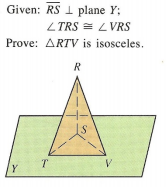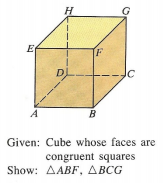3.5 In addition to Score 3.0 performance, the student demonstrates in-depth inferences and applications regarding the more complex content with partial success. Proficient “The Standard.” The student demonstrates no major errors or omissions regarding any of the information and processes that were end of instruction expectations.   The student can perform all the actions for each concept listed below: The student will be able to prove triangles are congruent. The student will be able to explain and apply the criteria of SSS, SAS, ASA, AAS, and HL to prove triangle congruency.Jennifer states that ∆ABC ≅ ∆DEF can be proven by either ASA or AAS.  Joshua disagrees – he says it can only be done by ASA because in ∆ABC it is missing the matching symbol to ∠D so we do not know if ∠A ≅ ∠D.  Who is correct?  Explain. 2.5 The student demonstrates no major errors or omissions regarding the simpler details and processes (Score 2.0 content) and partial knowledge of the more complex ideas and processes (Score 3.0 content). Progressing The student demonstrates no major errors or omissions regarding the simpler details and processes but exhibits major errors or omissions regarding the more complex ideas and processes (Score 3.0 content). The student can recognize and recall terminology to answer questions.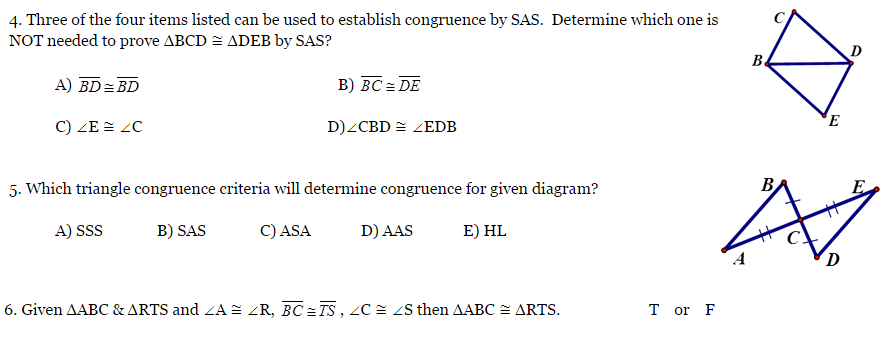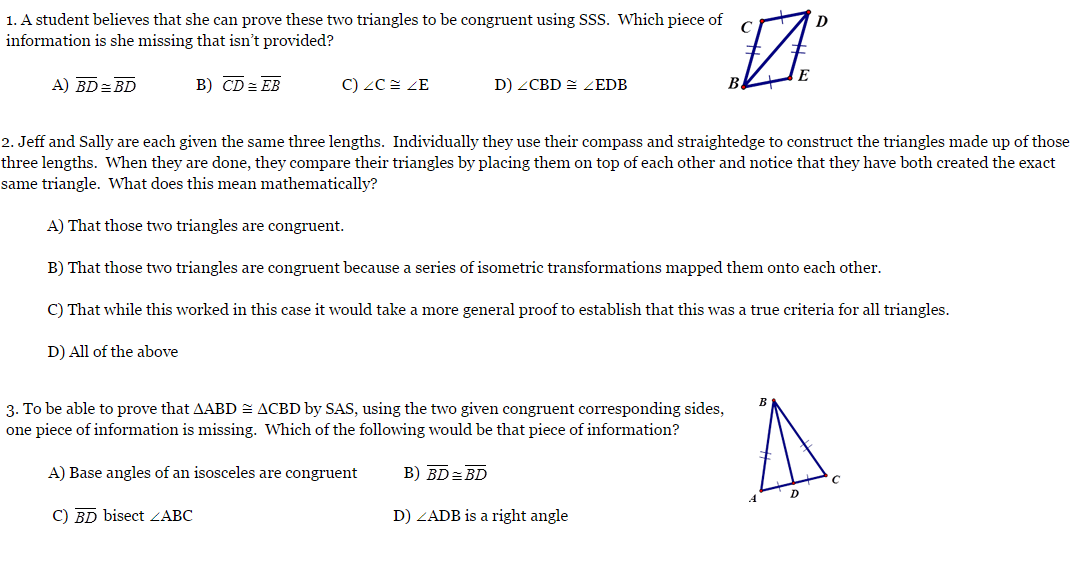1.5 The student demonstrates partial knowledge of the simpler details and processes (Score 2.0 content) but exhibits major errors or omissions regarding the more complex ideas and procedures (Score 3.0 content). Beginning With help, the student demonstrates a partial understanding of some of the simpler details and processes (Score 2.0 content) and some of the more complex ideas and processes (Score 3.0 content). - 0.5 With help, the student demonstrates a partial understanding of some of the simpler details and processes (Score 2.0 content) but not the more complex ideas and processes (Score 3.0 content). 0.0 Even with help, the student demonstrates no understanding or skill. -

## Resources

 Web Vocab

#### MAT-HS.G-CO.09

 MAT-HS Targeted Standards(G) Concept: Geometry(CO) Domain: CongruenceCluster: Prove geometric theorems MAT-HS.G-CO.09 Prove and apply theorems about lines and angles. Theorems include: vertical angles are congruent; when a transversal crosses parallel lines, alternate interior angles are congruent and corresponding angles are congruent; points on a perpendicular bisector of a line segment are exactly those equidistant from the segment’s endpoints.

## Student Learning Targets:

• I can
• I can

• I can
• I can

### Skills (Performance) Targets

• I can prove theorems pertaining to lines and angles.
• I can prove vertical angles are congruent.
• I can prove when a transversal crosses parallel lines, alternate interior angles are congruent and corresponding angles are congruent.
• I can prove points on a perpendicular bisector of a line segment are exactly those equidistant from the segment’s endpoints.

• I can
• I can

## Proficiency Scale

 Score Description Sample Activity 4.0 In addition to Score 3.0, the student demonstrates in-depth inferences and applications regarding more complex material that go beyond end of instruction expectations. - 3.5 In addition to Score 3.0 performance, the student demonstrates in-depth inferences and applications regarding the more complex content with partial success. 3.0 “The Standard.” The student demonstrates no major errors or omissions regarding any of the information and processes that were end of instruction expectations. - 2.5 The student demonstrates no major errors or omissions regarding the simpler details and processes (Score 2.0 content) and partial knowledge of the more complex ideas and processes (Score 3.0 content). 2.0 The student demonstrates no major errors or omissions regarding the simpler details and processes but exhibits major errors or omissions regarding the more complex ideas and processes (Score 3.0 content). - 1.5 The student demonstrates partial knowledge of the simpler details and processes (Score 2.0 content) but exhibits major errors or omissions regarding the more complex ideas and procedures (Score 3.0 content). 1.0 With help, the student demonstrates a partial understanding of some of the simpler details and processes (Score 2.0 content) and some of the more complex ideas and processes (Score 3.0 content). - 0.5 With help, the student demonstrates a partial understanding of some of the simpler details and processes (Score 2.0 content) but not the more complex ideas and processes (Score 3.0 content). 0.0 Even with help, the student demonstrates no understanding or skill. -

## Resources

 Web Vocab

#### MAT-HS.G-CO.09.a

 MAT-HS Targeted Standards(G) Concept: Geometry(CO) Domain: CongruenceCluster: Prove geometric theorems MAT-HS.G-CO.09.a Prove theorems about lines and angles. Theorems include but are not limited to: vertical angles are congruent; when a transversal crosses parallel lines, alternate interior angles are congruent and corresponding angles are congruent; points on a perpendicular bisector of a line segment are exactly those equidistant from the segment’s endpoints.

• I can
• I can

• I can
• I can

• I can
• I can

• I can
• I can

## Proficiency Scale

 Score Description Sample Activity Advanced In addition to Score 3.0, the student demonstrates in-depth inferences and applications regarding more complex material that go beyond end of instruction expectations. Error analysis (find errors in a given proof, fix the error and explain why it was incorrect) Concepts: Vertical Angles Angles created by parallel lines and a transversal Perpendicular Bisector theorems - 3.5 In addition to Score 3.0 performance, the student demonstrates in-depth inferences and applications regarding the more complex content with partial success. Proficient “The Standard.” The student demonstrates no major errors or omissions regarding any of the information and processes that were end of instruction expectations. Identify relevant given information Arrange reasoning in a logical sequence Support reasoning using appropriate justifications (theorems, postulates, definitions, etc.) Concepts: Vertical Angles Angles created by parallel lines and a transversal Perpendicular Bisector theorems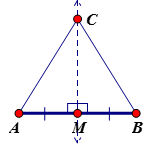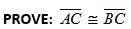2.5 The student demonstrates no major errors or omissions regarding the simpler details and processes (Score 2.0 content) and partial knowledge of the more complex ideas and processes (Score 3.0 content). Progressing The student demonstrates no major errors or omissions regarding the simpler details and processes but exhibits major errors or omissions regarding the more complex ideas and processes (Score 3.0 content). partially prove but reasoning has gaps, steps missing, incorrect reasoning, etc. recall theorems, definitions, and postulates fill out justification given a statement Concepts: Vertical Angles Angles created by parallel lines and a transversal Perpendicular Bisector theorems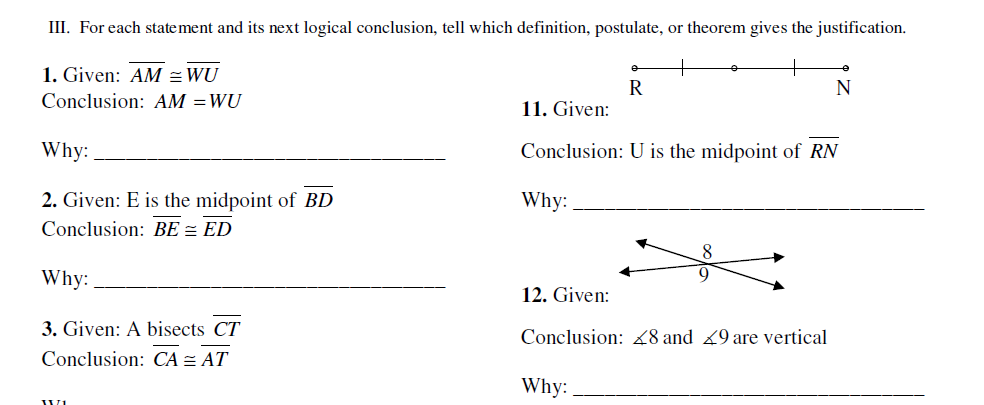1.5 The student demonstrates partial knowledge of the simpler details and processes (Score 2.0 content) but exhibits major errors or omissions regarding the more complex ideas and procedures (Score 3.0 content). Beginning With help, the student demonstrates a partial understanding of some of the simpler details and processes (Score 2.0 content) and some of the more complex ideas and processes (Score 3.0 content). - 0.5 With help, the student demonstrates a partial understanding of some of the simpler details and processes (Score 2.0 content) but not the more complex ideas and processes (Score 3.0 content). 0.0 Even with help, the student demonstrates no understanding or skill. -

## Resources

 Web Vocab

#### MAT-HS.G-CO.09.b

 MAT-HS Targeted Standards(G) Concept: Geometry(CO) Domain: CongruenceCluster: Prove geometric theorems MAT-HS.G-CO.09.b Apply theorems about lines and angles. Theorems include: vertical angles are congruent; when a transversal crosses parallel lines, alternate interior angles are congruent and corresponding angles are congruent; points on a perpendicular bisector of a line segment are exactly those equidistant from the segment’s endpoints. (Focus is on applying the theorems for C-CO.9B)

• I can
• I can

• I can
• I can

• I can
• I can

• I can
• I can

## Proficiency Scale

 Score Description Sample Activity Advanced In addition to Score 3.0, the student demonstrates in-depth inferences and applications regarding more complex material that go beyond end of instruction expectations. Concepts: Vertical Angles Angles created by parallel lines and a transversal Perpendicular Bisector theorems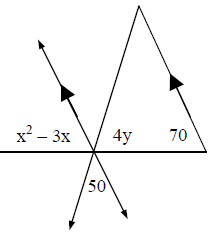Solve for x and y. 3.5 In addition to Score 3.0 performance, the student demonstrates in-depth inferences and applications regarding the more complex content with partial success. Proficient “The Standard.” The student demonstrates no major errors or omissions regarding any of the information and processes that were end of instruction expectations. Identify the relevant information Apply geometric relationships to create an algebraic model for the situation Solve and answer the given question (Ex. Solve for the given variable, Find the angle measurement) Concepts: Vertical Angles Angles created by parallel lines and a transversal Perpendicular Bisector theorem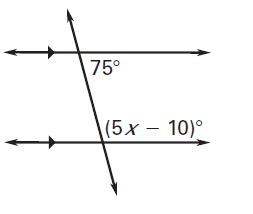Solve for x.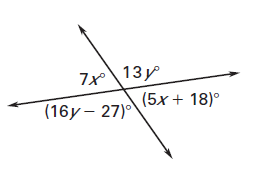Solve for x and y. 2.5 The student demonstrates no major errors or omissions regarding the simpler details and processes (Score 2.0 content) and partial knowledge of the more complex ideas and processes (Score 3.0 content). Progressing The student demonstrates no major errors or omissions regarding the simpler details and processes but exhibits major errors or omissions regarding the more complex ideas and processes (Score 3.0 content). can recognize and recall angle pairs can apply postulates, definitions, properties, and theorems to identify angle measurements Concepts: Vertical Angles Angles created by parallel lines and a transversal Perpendicular Bisector theorems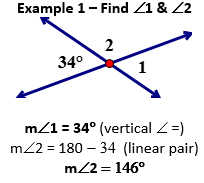Example 2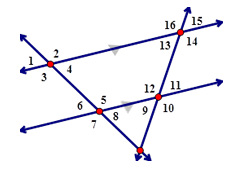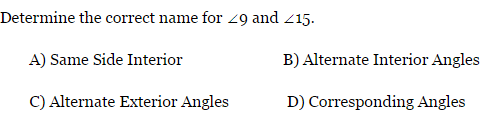1.5 The student demonstrates partial knowledge of the simpler details and processes (Score 2.0 content) but exhibits major errors or omissions regarding the more complex ideas and procedures (Score 3.0 content). Beginning With help, the student demonstrates a partial understanding of some of the simpler details and processes (Score 2.0 content) and some of the more complex ideas and processes (Score 3.0 content). - 0.5 With help, the student demonstrates a partial understanding of some of the simpler details and processes (Score 2.0 content) but not the more complex ideas and processes (Score 3.0 content). 0.0 Even with help, the student demonstrates no understanding or skill. -

## Resources

 Web Vocab

#### MAT-HS.G-CO.10

 MAT-HS Targeted Standards(G) Concept: Geometry(CO) Domain: CongruenceCluster: Prove geometric theorems MAT-HS.G-CO.10 Prove and apply theorems about triangles. Theorems include: measures of interior angles of a triangle sum to 180°; base angles of isosceles triangles are congruent; the segment joining midpoints of two sides of a triangle is parallel to the third side and half the length; the medians of a triangle meet at a point.

## Student Learning Targets:

• I can
• I can

• I can
• I can

### Skills (Performance) Targets

• I can prove theorems about triangles.
• I can prove the measures of interior angles of a triangle have a sum of 180°.
• I can prove base angles of isosceles triangles are congruent.
• I can prove the segment joining midpoints of two sides of a triangle is parallel to the third side and half the length.
• I can prove the medians of a triangle meet at a point.

• I can
• I can

## Proficiency Scale

 Score Description Sample Activity 4.0 In addition to Score 3.0, the student demonstrates in-depth inferences and applications regarding more complex material that go beyond end of instruction expectations. - 3.5 In addition to Score 3.0 performance, the student demonstrates in-depth inferences and applications regarding the more complex content with partial success. 3.0 “The Standard.” The student demonstrates no major errors or omissions regarding any of the information and processes that were end of instruction expectations. - 2.5 The student demonstrates no major errors or omissions regarding the simpler details and processes (Score 2.0 content) and partial knowledge of the more complex ideas and processes (Score 3.0 content). 2.0 The student demonstrates no major errors or omissions regarding the simpler details and processes but exhibits major errors or omissions regarding the more complex ideas and processes (Score 3.0 content). - 1.5 The student demonstrates partial knowledge of the simpler details and processes (Score 2.0 content) but exhibits major errors or omissions regarding the more complex ideas and procedures (Score 3.0 content). 1.0 With help, the student demonstrates a partial understanding of some of the simpler details and processes (Score 2.0 content) and some of the more complex ideas and processes (Score 3.0 content). - 0.5 With help, the student demonstrates a partial understanding of some of the simpler details and processes (Score 2.0 content) but not the more complex ideas and processes (Score 3.0 content). 0.0 Even with help, the student demonstrates no understanding or skill. -

## Resources

 Web Vocab

#### MAT-HS.G-CO.10.a

 MAT-HS Targeted Standards(G) Concept: Geometry(CO) Domain: CongruenceCluster: Prove geometric theorems MAT-HS.G-CO.10.a Prove theorems about triangles. (Theorems include but are not limited to: Measures of interior angles of a triangle sum to 180 degrees; base angles of isosceles triangles are congruent; the segment joining midpoints of two sides of a triangle is parallel to the third side and half the length; the medians of a triangle meet at a point.) (Focus on proving for this proficiency scale)

• I can
• I can

• I can
• I can

• I can
• I can

• I can
• I can

## Proficiency Scale

 Score Description Sample Activity Advanced In addition to Score 3.0, the student demonstrates in-depth inferences and applications regarding more complex material that go beyond end of instruction expectations. Analyze a proof with errors and make corrections - 3.5 In addition to Score 3.0 performance, the student demonstrates in-depth inferences and applications regarding the more complex content with partial success. Proficient “The Standard.” The student demonstrates no major errors or omissions regarding any of the information and processes that were end of instruction expectations.   Identify relevant given information Arrange reasoning in a logical sequence Support reasoning using appropriate justifications (theorems, postulates, definitions, etc.) Midsegment of a Triangle Proof Triangle Interior Angle Proof Prove a Triangle is Isosceles 2.5 The student demonstrates no major errors or omissions regarding the simpler details and processes (Score 2.0 content) and partial knowledge of the more complex ideas and processes (Score 3.0 content). Progressing The student demonstrates no major errors or omissions regarding the simpler details and processes but exhibits major errors or omissions regarding the more complex ideas and processes (Score 3.0 content).   partially prove but reasoning has gaps, steps missing, incorrect reasoning, etc. recall theorems, definitions, and postulates fill out justification given a statement Given a statement, the student can fill out the justification.  (skeleton proof) 1.5 The student demonstrates partial knowledge of the simpler details and processes (Score 2.0 content) but exhibits major errors or omissions regarding the more complex ideas and procedures (Score 3.0 content). Beginning With help, the student demonstrates a partial understanding of some of the simpler details and processes (Score 2.0 content) and some of the more complex ideas and processes (Score 3.0 content). - 0.5 With help, the student demonstrates a partial understanding of some of the simpler details and processes (Score 2.0 content) but not the more complex ideas and processes (Score 3.0 content). 0.0 Even with help, the student demonstrates no understanding or skill. -

## Resources

 Web Vocab

#### MAT-HS.G-CO.10.b

 MAT-HS Targeted Standards(G) Concept: Geometry(CO) Domain: CongruenceCluster: Prove geometric theorems MAT-HS.G-CO.10.b Apply theorems about triangles. (Theorems include: measures of interior angles of a triangle sum to 180 degrees; base angles of isosceles triangles are congruent; the segment joining midpoints of two sides of a triangle is parallel to the third side and half the length; the medians of a triangle meet at a point. (Focus on Applying)

• I can
• I can

• I can
• I can

• I can
• I can

• I can
• I can

## Proficiency Scale

 Score Description Sample Activity Advanced In addition to Score 3.0, the student demonstrates in-depth inferences and applications regarding more complex material that go beyond end of instruction expectations. Analyze a solution and correct the errors Investigate and provide multiple methods for finding a solution to a given problem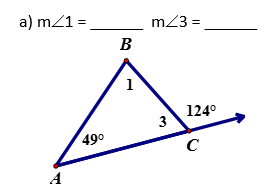Find the measures of these angles in two different ways. Explain the steps you took for each method. 3.5 In addition to Score 3.0 performance, the student demonstrates in-depth inferences and applications regarding the more complex content with partial success. Proficient “The Standard.” The student demonstrates no major errors or omissions regarding any of the information and processes that were end of instruction expectations. Apply theorems (postulates, definitions, etc.) about triangles to solve problems Justify how to set up a problem using theorems (postulates, definitions, etc.) about triangles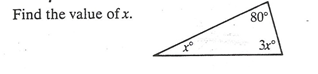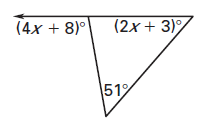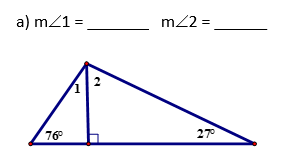2.5 The student demonstrates no major errors or omissions regarding the simpler details and processes (Score 2.0 content) and partial knowledge of the more complex ideas and processes (Score 3.0 content). Progressing The student demonstrates no major errors or omissions regarding the simpler details and processes but exhibits major errors or omissions regarding the more complex ideas and processes (Score 3.0 content).   Identify or relate the theorem used to set up the problem Define the vocabulary associated with triangle theorems Can identify the components of triangles - 1.5 The student demonstrates partial knowledge of the simpler details and processes (Score 2.0 content) but exhibits major errors or omissions regarding the more complex ideas and procedures (Score 3.0 content). Beginning With help, the student demonstrates a partial understanding of some of the simpler details and processes (Score 2.0 content) and some of the more complex ideas and processes (Score 3.0 content). - 0.5 With help, the student demonstrates a partial understanding of some of the simpler details and processes (Score 2.0 content) but not the more complex ideas and processes (Score 3.0 content). 0.0 Even with help, the student demonstrates no understanding or skill. -

## Resources

 Web Vocab

#### MAT-HS.G-CO.11

 MAT-HS Targeted Standards(G) Concept: Geometry(CO) Domain: CongruenceCluster: Prove geometric theorems MAT-HS.G-CO.11 Prove and apply theorems about parallelograms. Theorems include: opposite sides are congruent, opposite angles are congruent, the diagonals of a parallelogram bisect each other, and conversely, rectangles are parallelograms with congruent diagonals.

## Student Learning Targets:

• I can
• I can

• I can
• I can

### Skills (Performance) Targets

• I can prove theorems about parallelograms.
• I can prove opposite sides are congruent.
• I can prove opposite angles are congruent.
• I can prove the diagonals of a parallelogram bisect each other.
• I can prove rectangles are parallelograms with congruent diagonals.

• I can
• I can

## Proficiency Scale

 Score Description Sample Activity 4.0 In addition to Score 3.0, the student demonstrates in-depth inferences and applications regarding more complex material that go beyond end of instruction expectations. - 3.5 In addition to Score 3.0 performance, the student demonstrates in-depth inferences and applications regarding the more complex content with partial success. 3.0 “The Standard.” The student demonstrates no major errors or omissions regarding any of the information and processes that were end of instruction expectations. - 2.5 The student demonstrates no major errors or omissions regarding the simpler details and processes (Score 2.0 content) and partial knowledge of the more complex ideas and processes (Score 3.0 content). 2.0 The student demonstrates no major errors or omissions regarding the simpler details and processes but exhibits major errors or omissions regarding the more complex ideas and processes (Score 3.0 content). - 1.5 The student demonstrates partial knowledge of the simpler details and processes (Score 2.0 content) but exhibits major errors or omissions regarding the more complex ideas and procedures (Score 3.0 content). 1.0 With help, the student demonstrates a partial understanding of some of the simpler details and processes (Score 2.0 content) and some of the more complex ideas and processes (Score 3.0 content). - 0.5 With help, the student demonstrates a partial understanding of some of the simpler details and processes (Score 2.0 content) but not the more complex ideas and processes (Score 3.0 content). 0.0 Even with help, the student demonstrates no understanding or skill. -

## Resources

 Web Vocab

#### MAT-HS.G-CO.11.a

 MAT-HS Targeted Standards(G) Concept: Geometry(CO) Domain: CongruenceCluster: Prove geometric theorems MAT-HS.G-CO.11.b Prove theorems about parallelograms. (Focus on Prove for G-CO.11) (Theorems include but are not limited to: opposite sides are congruent, opposite angles are congruent, the diagonals of a parallelogram bisect each other, and conversely, rectangles are parallelograms with congruent diagonals.)

• I can
• I can

• I can
• I can

• I can
• I can

• I can
• I can

## Proficiency Scale

 Score Description Sample Activity Advanced In addition to Score 3.0, the student demonstrates in-depth inferences and applications regarding more complex material that go beyond end of instruction expectations. Analyze a proof with errors and make corrections - 3.5 In addition to Score 3.0 performance, the student demonstrates in-depth inferences and applications regarding the more complex content with partial success. Proficient “The Standard.” The student demonstrates no major errors or omissions regarding any of the information and processes that were end of instruction expectations. The student can perform all the actions for each concept listed below: Identify relevant information Arrange reasoning in a logical sequence Support reasoning using appropriate justifications (theorems, postulates, definitions, etc.)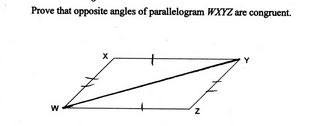2.5 The student demonstrates no major errors or omissions regarding the simpler details and processes (Score 2.0 content) and partial knowledge of the more complex ideas and processes (Score 3.0 content). Progressing The student demonstrates no major errors or omissions regarding the simpler details and processes but exhibits major errors or omissions regarding the more complex ideas and processes (Score 3.0 content). Partially prove but reasoning has gaps, steps missing, incorrect reasoning, etc. Recall theorems, definitions, and postulates Fill out justification given a statement - 1.5 The student demonstrates partial knowledge of the simpler details and processes (Score 2.0 content) but exhibits major errors or omissions regarding the more complex ideas and procedures (Score 3.0 content). Beginning With help, the student demonstrates a partial understanding of some of the simpler details and processes (Score 2.0 content) and some of the more complex ideas and processes (Score 3.0 content). - 0.5 With help, the student demonstrates a partial understanding of some of the simpler details and processes (Score 2.0 content) but not the more complex ideas and processes (Score 3.0 content). 0.0 Even with help, the student demonstrates no understanding or skill. -

## Resources

 Web Vocab

#### MAT-HS.G-CO.11.b

 MAT-HS Targeted Standards(G) Concept: Geometry(CO) Domain: CongruenceCluster: Prove geometric theorems MAT-HS.G-CO.11.b Apply theorems about parallelograms. Theorems include but are not limited to: opposite sides are congruent, opposite angles are congruent, the diagonals of a parallelogram bisect each other, and conversely, rectangles are parallelograms with congruent diagonals. (Focus is on Application for G-CO.11B)

• I can
• I can

• I can
• I can

• I can
• I can

• I can
• I can

## Proficiency Scale

 Score Description Sample Activity Advanced In addition to Score 3.0, the student demonstrates in-depth inferences and applications regarding more complex material that go beyond end of instruction expectations. - 3.5 In addition to Score 3.0 performance, the student demonstrates in-depth inferences and applications regarding the more complex content with partial success. Proficient “The Standard.” The student demonstrates no major errors or omissions regarding any of the information and processes that were end of instruction expectations. Apply theorems (postulates, definitions, etc.) about parallelograms to solve problems Justify how to set up a problem using theorems (postulates, definitions, etc.) about parallelograms Solve for x and y.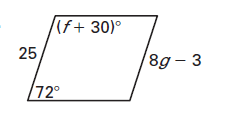2.5 The student demonstrates no major errors or omissions regarding the simpler details and processes (Score 2.0 content) and partial knowledge of the more complex ideas and processes (Score 3.0 content). Progressing The student demonstrates no major errors or omissions regarding the simpler details and processes but exhibits major errors or omissions regarding the more complex ideas and processes (Score 3.0 content). Identify the theorem, postulate, or definition used to set up and solve a problem Able to recognize and recall important vocabulary such as:                 opposite sides                 opposite angles                 diagonals                 congruent triangles                  perpendicular                 CPCTC Students are able to find the missing measurements  in a parallelogram given some basic information Can determine if a given figure is a parallelogram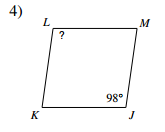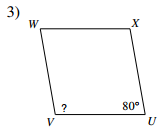Identify if the given figure is a parallelogram.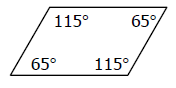1.5 The student demonstrates partial knowledge of the simpler details and processes (Score 2.0 content) but exhibits major errors or omissions regarding the more complex ideas and procedures (Score 3.0 content). Beginning With help, the student demonstrates a partial understanding of some of the simpler details and processes (Score 2.0 content) and some of the more complex ideas and processes (Score 3.0 content). - 0.5 With help, the student demonstrates a partial understanding of some of the simpler details and processes (Score 2.0 content) but not the more complex ideas and processes (Score 3.0 content). 0.0 Even with help, the student demonstrates no understanding or skill. -

## Resources

 Web Vocab

#### MAT-HS.G-CO.12

 MAT-HS Targeted Standards(G) Concept: Geometry(CO) Domain: CongruenceCluster: Make geometric constructions MAT-HS.G-CO.12 Make basic geometric constructions with a variety of tools and methods. Copying a segment; copying an angle; bisecting a segment; bisecting an angle; constructing perpendicular lines, including the perpendicular bisector of a line segment; and constructing a line parallel to a given line through a point not on the line.  Tools may include compass and straightedge, string, reflective devices, paper folding or dynamic geometric software.

## Student Learning Targets:

• I can
• I can

• I can
• I can

### Skills (Performance) Targets

• I can use a variety of methods and tools to:
• copy a segment
• copy an angle
• bisect a segment
• bisect an angle
• construct perpendicular
• lines, including the perpendicular bisector of a line segment
• construct a line parallel to a given line through a point not on the line

• I can
• I can

## Proficiency Scale

 Score Description Sample Activity Advanced In addition to Score 3.0, the student demonstrates in-depth inferences and applications regarding more complex material that go beyond end of instruction expectations. Explain how to construct a geometric figure Explain the type of construction and the next step in completing the construction, giving specific steps.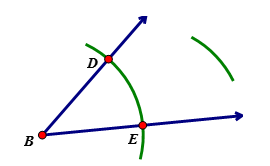3.5 In addition to Score 3.0 performance, the student demonstrates in-depth inferences and applications regarding the more complex content with partial success. Proficient “The Standard.” The student demonstrates no major errors or omissions regarding any of the information and processes that were end of instruction expectations. Construct a geometric figure using various tools and/or methods  (Ex: compass and straightedge, Geogebra) (a) copy a given line segment   (b) copy an angle. (c) bisect a line segment. (d) bisect an angle. (e) construct a line perpendicular to a given line through a point on that line. (f) construct a line perpendicular to a given line through a point not on that line. (g) construct the perpendicular bisector of a line segment. (h) construct a line parallel to a given line through a point not on the line.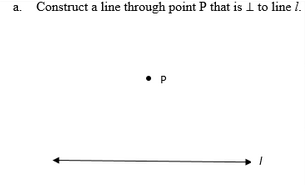Identify and complete the construction.2.5 The student demonstrates no major errors or omissions regarding the simpler details and processes (Score 2.0 content) and partial knowledge of the more complex ideas and processes (Score 3.0 content). Progressing The student demonstrates no major errors or omissions regarding the simpler details and processes but exhibits major errors or omissions regarding the more complex ideas and processes (Score 3.0 content). can recognize and recall terminology to answer questions  identify the type of construction given an example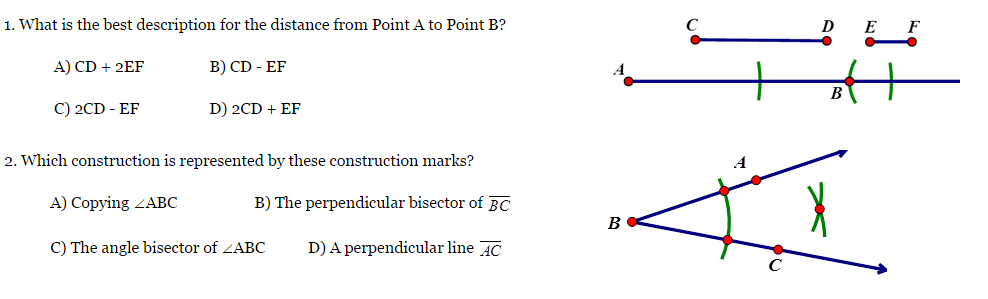1.5 The student demonstrates partial knowledge of the simpler details and processes (Score 2.0 content) but exhibits major errors or omissions regarding the more complex ideas and procedures (Score 3.0 content). Beginning With help, the student demonstrates a partial understanding of some of the simpler details and processes (Score 2.0 content) and some of the more complex ideas and processes (Score 3.0 content). - 0.5 With help, the student demonstrates a partial understanding of some of the simpler details and processes (Score 2.0 content) but not the more complex ideas and processes (Score 3.0 content). 0.0 Even with help, the student demonstrates no understanding or skill. -

## Resources

 Web Vocab

#### MAT-HS.G-CO.13

 MAT-HS Targeted Standards(G) Concept: Geometry(CO) Domain: CongruenceCluster: Make geometric constructions MAT-HS.G-CO.13 Construct an equilateral triangle, a square, and a regular hexagon inscribed in a circle.

## Student Learning Targets:

• I can
• I can

• I can
• I can

### Skills (Performance) Targets

• I can construct an equilateral triangle inscribed in a circle.
• I can construct a square inscribed in a circle.
• I can construct a regular hexagon inscribed in a circle.

• I can
• I can

## Proficiency Scale

 Score Description Sample Activity 4.0 In addition to Score 3.0, the student demonstrates in-depth inferences and applications regarding more complex material that go beyond end of instruction expectations. - 3.5 In addition to Score 3.0 performance, the student demonstrates in-depth inferences and applications regarding the more complex content with partial success. 3.0 “The Standard.” The student demonstrates no major errors or omissions regarding any of the information and processes that were end of instruction expectations. - 2.5 The student demonstrates no major errors or omissions regarding the simpler details and processes (Score 2.0 content) and partial knowledge of the more complex ideas and processes (Score 3.0 content). 2.0 The student demonstrates no major errors or omissions regarding the simpler details and processes but exhibits major errors or omissions regarding the more complex ideas and processes (Score 3.0 content). - 1.5 The student demonstrates partial knowledge of the simpler details and processes (Score 2.0 content) but exhibits major errors or omissions regarding the more complex ideas and procedures (Score 3.0 content). 1.0 With help, the student demonstrates a partial understanding of some of the simpler details and processes (Score 2.0 content) and some of the more complex ideas and processes (Score 3.0 content). - 0.5 With help, the student demonstrates a partial understanding of some of the simpler details and processes (Score 2.0 content) but not the more complex ideas and processes (Score 3.0 content). 0.0 Even with help, the student demonstrates no understanding or skill. -

## Resources

 Web Vocab

#### MAT-HS.G-GMD.01

 MAT-HS Targeted Standards(G) Concept: Geometry(GMD) Domain: Geometric Measurement and DimensionCluster: Explain volume formulas and use them to solve problems. MAT-HS.G-GMD.01 Give an informal argument for the formulas for the circumference of a circle, area of a circle, volume of a cylinder, pyramid, and cone. Use dissection arguments, Cavalieri’s principle, and informal limit arguments.

## Student Learning Targets:

• I can
• I can

### Reasoning Targets

• I can explain the formulas for circumference of a circle and area of a circle by determining the meaning of each term.
• I can explain the formulas for volume of a cylinder, pyramid and cone by determining the meaning of each term.

• I can
• I can

• I can
• I can

## Proficiency Scale

 Score Description Sample Activity 4.0 In addition to Score 3.0, the student demonstrates in-depth inferences and applications regarding more complex material that go beyond end of instruction expectations. - 3.5 In addition to Score 3.0 performance, the student demonstrates in-depth inferences and applications regarding the more complex content with partial success. 3.0 “The Standard.” The student demonstrates no major errors or omissions regarding any of the information and processes that were end of instruction expectations. - 2.5 The student demonstrates no major errors or omissions regarding the simpler details and processes (Score 2.0 content) and partial knowledge of the more complex ideas and processes (Score 3.0 content). 2.0 The student demonstrates no major errors or omissions regarding the simpler details and processes but exhibits major errors or omissions regarding the more complex ideas and processes (Score 3.0 content). - 1.5 The student demonstrates partial knowledge of the simpler details and processes (Score 2.0 content) but exhibits major errors or omissions regarding the more complex ideas and procedures (Score 3.0 content). 1.0 With help, the student demonstrates a partial understanding of some of the simpler details and processes (Score 2.0 content) and some of the more complex ideas and processes (Score 3.0 content). - 0.5 With help, the student demonstrates a partial understanding of some of the simpler details and processes (Score 2.0 content) but not the more complex ideas and processes (Score 3.0 content). 0.0 Even with help, the student demonstrates no understanding or skill. -

## Resources

 Web Vocab

#### MAT-HS.G-GMD.02

 MAT-HS Targeted Standards(G) Concept: Geometry(GMD) Domain: Geometric Measurement and DimensionCluster: Explain volume formulas and use them to solve problems.  MAT-HS.G-GMD.02 Calculate the surface area for prisms, cylinders, pyramids, cones, and spheres to solve problems.

• I can
• I can

• I can
• I can

• I can
• I can

• I can
• I can

## Proficiency Scale

 Score Description Sample Activity Advanced In addition to Score 3.0, the student demonstrates in-depth inferences and applications regarding more complex material that go beyond end of instruction expectations. Create a solid of revolution by rotating a 2 dimensional figure about a line and find the surface area of that solid. A geometric figure is created when bounded by the lines y=¾ x-3, y=0, x=0.  Draw a picture of the region being revolved about the x-axis.  Determine the surface area of the geometric solid. 3.5 In addition to Score 3.0 performance, the student demonstrates in-depth inferences and applications regarding the more complex content with partial success. Proficient “The Standard.” The student demonstrates no major errors or omissions regarding any of the information and processes that were end of instruction expectations. Calculate the surface area formulas for cylinders, pyramids, cones, and spheres to solve problems Solve for various heights and lengths for use in the surface area formulas using inductive reasoning, pythagorean theorem, and trigonometry. Label the answer using the correct units based on the context of the problem. Give either the approximate or exact answer, given the context of the problem. Find the surface area of the figure: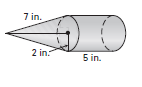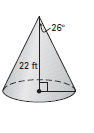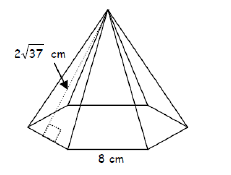2.5 The student demonstrates no major errors or omissions regarding the simpler details and processes (Score 2.0 content) and partial knowledge of the more complex ideas and processes (Score 3.0 content). Progressing The student demonstrates no major errors or omissions regarding the simpler details and processes but exhibits major errors or omissions regarding the more complex ideas and processes (Score 3.0 content). Select the appropriate formula for finding the surface area of a solid Students can solve for surface area of given shapes when given all necessary measurements Find the surface area of the figure: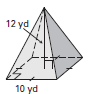1.5 The student demonstrates partial knowledge of the simpler details and processes (Score 2.0 content) but exhibits major errors or omissions regarding the more complex ideas and procedures (Score 3.0 content). Beginning With help, the student demonstrates a partial understanding of some of the simpler details and processes (Score 2.0 content) and some of the more complex ideas and processes (Score 3.0 content). can solve for surface area with help - 0.5 With help, the student demonstrates a partial understanding of some of the simpler details and processes (Score 2.0 content) but not the more complex ideas and processes (Score 3.0 content). 0.0 Even with help, the student demonstrates no understanding or skill. -

## Resources

 Web Vocab

#### MAT-HS.G-GMD.03

 MAT-HS Targeted Standards(G) Concept: Geometry(GMD) Domain: Geometric Measurement and DimensionCluster: Explain volume formulas and use them to solve problems MAT-HS.G-GMD.03 Know and apply volume formulas for prisms, cylinders, pyramids, cones, and spheres to solve problems.

## Student Learning Targets:

### Knowledge Targets

• I can choose the appropriate formula from a list.

### Reasoning Targets

• I can, given formulas, compute the surface area and volume of a right prism, right cylinder, right pyramid, right cone, and sphere.

• I can
• I can

• I can
• I can

## Proficiency Scale

 Score Description Sample Activity Advanced In addition to Score 3.0, the student demonstrates in-depth inferences and applications regarding more complex material that go beyond end of instruction expectations. Create a solid of revolution by rotating a 2 dimensional figure about a line and find the volume of that solid. A geometric figure is created when bounded by the lines y=¾ x-3, y=0, x=0.  Draw a picture of the region being revolved about the x-axis.  Determine the volume of the geometric solid. 3.5 In addition to Score 3.0 performance, the student demonstrates in-depth inferences and applications regarding the more complex content with partial success. Proficient “The Standard.” The student demonstrates no major errors or omissions regarding any of the information and processes that were end of instruction expectations. Know and use volume formulas for cylinders, pyramids, cones, and spheres to solve problems. Solve for various heights and lengths for use in the volume formulas using inductive reasoning, pythagorean theorem, and trigonometry. Label the answer using the correct units based on the context of the problem. Give either the approximate or exact answer, given the context of the problem. Find the volume of the figure:Find the value of x.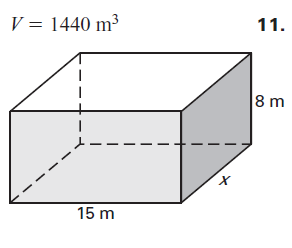2.5 The student demonstrates no major errors or omissions regarding the simpler details and processes (Score 2.0 content) and partial knowledge of the more complex ideas and processes (Score 3.0 content). Progressing The student demonstrates no major errors or omissions regarding the simpler details and processes but exhibits major errors or omissions regarding the more complex ideas and processes (Score 3.0 content). Select the appropriate formula for finding the volume of a solid. Students can solve for volume of given shapes when given all necessary measurements Find the volume of the figure:1.5 The student demonstrates partial knowledge of the simpler details and processes (Score 2.0 content) but exhibits major errors or omissions regarding the more complex ideas and procedures (Score 3.0 content). Beginning With help, the student demonstrates a partial understanding of some of the simpler details and processes (Score 2.0 content) and some of the more complex ideas and processes (Score 3.0 content). Students can solve for volume with help. 0.5 With help, the student demonstrates a partial understanding of some of the simpler details and processes (Score 2.0 content) but not the more complex ideas and processes (Score 3.0 content). 0.0 Even with help, the student demonstrates no understanding or skill. -

## Resources

 Web Vocab

#### MAT-HS.G-GMD.04

 MAT-HS Targeted Standards(G) Concept: Geometry(GMD) Domain: Geometric Measurement and DimensionCluster: Visualize relationships between two-dimensional and three-dimensional objects MAT-HS.G-GMD.04 Identify the shapes of two-dimensional cross-sections of three-dimensional objects, and identify three-dimensional objects generated by rotations of two-dimensional objects.

## Student Learning Targets:

### Knowledge Targets

• I can identify the properties and attributes of two and three-dimensional objects that distinguish one from another; e.g., a square is a parallelogram with four congruent sides and four right angles; a cylinder has two parallel circular bases.
• I can identify two dimensional cross sections of three dimensional objects.
• I can identify three dimensional objects created by rotating two dimensional objects.

• I can
• I can

• I can
• I can

• I can
• I can

## Proficiency Scale

 Score Description Sample Activity 4.0 In addition to Score 3.0, the student demonstrates in-depth inferences and applications regarding more complex material that go beyond end of instruction expectations. - 3.5 In addition to Score 3.0 performance, the student demonstrates in-depth inferences and applications regarding the more complex content with partial success. 3.0 “The Standard.” The student demonstrates no major errors or omissions regarding any of the information and processes that were end of instruction expectations. - 2.5 The student demonstrates no major errors or omissions regarding the simpler details and processes (Score 2.0 content) and partial knowledge of the more complex ideas and processes (Score 3.0 content). 2.0 The student demonstrates no major errors or omissions regarding the simpler details and processes but exhibits major errors or omissions regarding the more complex ideas and processes (Score 3.0 content). - 1.5 The student demonstrates partial knowledge of the simpler details and processes (Score 2.0 content) but exhibits major errors or omissions regarding the more complex ideas and procedures (Score 3.0 content). 1.0 With help, the student demonstrates a partial understanding of some of the simpler details and processes (Score 2.0 content) and some of the more complex ideas and processes (Score 3.0 content). - 0.5 With help, the student demonstrates a partial understanding of some of the simpler details and processes (Score 2.0 content) but not the more complex ideas and processes (Score 3.0 content). 0.0 Even with help, the student demonstrates no understanding or skill. -

## Resources

 Web Vocab

#### MAT-HS.G-GPE.01

 MAT-HS Targeted Standards(G) Concept: Geometry(GPE) Domain: Expressing Geometric Properties with EquationsCluster: Translate between the geometric description and the equation for a conic section MAT-HS.G-GPE.01 Derive the equation of a circle of given center and radius using the Pythagorean Theorem; complete the square to find the center and radius of a circle given by an equation.

## Student Learning Targets:

• I can
• I can

• I can
• I can

### Skills (Performance) Targets

• I can use the Pythagorean Theorem to derive the equation of a circle, given the center and radius. I can given an equation of a circle, complete the square to find the center and radius.

• I can
• I can

## Proficiency Scale

 Score Description Sample Activity 4.0 In addition to Score 3.0, the student demonstrates in-depth inferences and applications regarding more complex material that go beyond end of instruction expectations. - 3.5 In addition to Score 3.0 performance, the student demonstrates in-depth inferences and applications regarding the more complex content with partial success. 3.0 “The Standard.” The student demonstrates no major errors or omissions regarding any of the information and processes that were end of instruction expectations. - 2.5 The student demonstrates no major errors or omissions regarding the simpler details and processes (Score 2.0 content) and partial knowledge of the more complex ideas and processes (Score 3.0 content). 2.0 The student demonstrates no major errors or omissions regarding the simpler details and processes but exhibits major errors or omissions regarding the more complex ideas and processes (Score 3.0 content). - 1.5 The student demonstrates partial knowledge of the simpler details and processes (Score 2.0 content) but exhibits major errors or omissions regarding the more complex ideas and procedures (Score 3.0 content). 1.0 With help, the student demonstrates a partial understanding of some of the simpler details and processes (Score 2.0 content) and some of the more complex ideas and processes (Score 3.0 content). - 0.5 With help, the student demonstrates a partial understanding of some of the simpler details and processes (Score 2.0 content) but not the more complex ideas and processes (Score 3.0 content). 0.0 Even with help, the student demonstrates no understanding or skill. -

## Resources

 Web Vocab

#### MAT-HS.G-GPE.02

 MAT-HS Targeted Standards(G) Concept: Geometry(GPE) Domain: Expressing Geometric Properties with EquationsCluster: Translate between the geometric description and the equation for a conic section MAT-HS.G-GPE.02 Derive the equation of a parabola given a focus and directrix.

## Student Learning Targets:

### Knowledge Targets

• I can, given a parabola, identify the vertex, focus, directrix, and axis of symmetry, noting that every point on the parabola is equidistant from the focus and the directrix.

• I can
• I can

### Skills (Performance) Targets

• I can, given a focus and directrix, derive the equation of a parabola.

• I can
• I can

## Proficiency Scale

 Score Description Sample Activity 4.0 In addition to Score 3.0, the student demonstrates in-depth inferences and applications regarding more complex material that go beyond end of instruction expectations. - 3.5 In addition to Score 3.0 performance, the student demonstrates in-depth inferences and applications regarding the more complex content with partial success. 3.0 “The Standard.” The student demonstrates no major errors or omissions regarding any of the information and processes that were end of instruction expectations. - 2.5 The student demonstrates no major errors or omissions regarding the simpler details and processes (Score 2.0 content) and partial knowledge of the more complex ideas and processes (Score 3.0 content). 2.0 The student demonstrates no major errors or omissions regarding the simpler details and processes but exhibits major errors or omissions regarding the more complex ideas and processes (Score 3.0 content). - 1.5 The student demonstrates partial knowledge of the simpler details and processes (Score 2.0 content) but exhibits major errors or omissions regarding the more complex ideas and procedures (Score 3.0 content). 1.0 With help, the student demonstrates a partial understanding of some of the simpler details and processes (Score 2.0 content) and some of the more complex ideas and processes (Score 3.0 content). - 0.5 With help, the student demonstrates a partial understanding of some of the simpler details and processes (Score 2.0 content) but not the more complex ideas and processes (Score 3.0 content). 0.0 Even with help, the student demonstrates no understanding or skill. -

## Resources

 Web Vocab

#### MAT-HS.G-GPE.03

 MAT-HS Targeted Standards(G) Concept: Geometry(GPE) Domain: Expressing Geometric Properties with EquationsCluster: Translate between the geometric description and the equation for a conic section. MAT-HS.G-GPE.03 Derive the equations of ellipses and hyperbol as given foci, using the fact that the sum or difference of distances from the foci is constant.

• I can
• I can

• I can
• I can

• I can
• I can

• I can
• I can

## Proficiency Scale

 Score Description Sample Activity 4.0 In addition to Score 3.0, the student demonstrates in-depth inferences and applications regarding more complex material that go beyond end of instruction expectations. - 3.5 In addition to Score 3.0 performance, the student demonstrates in-depth inferences and applications regarding the more complex content with partial success. 3.0 “The Standard.” The student demonstrates no major errors or omissions regarding any of the information and processes that were end of instruction expectations. - 2.5 The student demonstrates no major errors or omissions regarding the simpler details and processes (Score 2.0 content) and partial knowledge of the more complex ideas and processes (Score 3.0 content). 2.0 The student demonstrates no major errors or omissions regarding the simpler details and processes but exhibits major errors or omissions regarding the more complex ideas and processes (Score 3.0 content). - 1.5 The student demonstrates partial knowledge of the simpler details and processes (Score 2.0 content) but exhibits major errors or omissions regarding the more complex ideas and procedures (Score 3.0 content). 1.0 With help, the student demonstrates a partial understanding of some of the simpler details and processes (Score 2.0 content) and some of the more complex ideas and processes (Score 3.0 content). - 0.5 With help, the student demonstrates a partial understanding of some of the simpler details and processes (Score 2.0 content) but not the more complex ideas and processes (Score 3.0 content). 0.0 Even with help, the student demonstrates no understanding or skill. -

## Resources

 Web Vocab

#### MAT-HS.G-GPE.04

 MAT-HS Targeted Standards(G) Concept: Geometry(GPE) Domain: Expressing Geometric Properties with EquationsCluster: Use coordinates to prove simple geometric theorems algebraically MAT-HS.G-GPE.04 Use coordinates to prove simple geometric theorems algebraically. For example, prove or disprove that a figure defined by four given points in the coordinate plane is a rectangle; prove or disprove that the point (1, √3) lies on the circle centered at the origin and containing the point (0, 2).

## Student Learning Targets:

• I can
• I can

• I can
• I can

### Skills (Performance) Targets

• I can use coordinate geometry to prove geometric theorems algebraically.

• I can
• I can

## Proficiency Scale

 Score Description Sample Activity 4.0 In addition to Score 3.0, the student demonstrates in-depth inferences and applications regarding more complex material that go beyond end of instruction expectations. - 3.5 In addition to Score 3.0 performance, the student demonstrates in-depth inferences and applications regarding the more complex content with partial success. 3.0 “The Standard.” The student demonstrates no major errors or omissions regarding any of the information and processes that were end of instruction expectations. - 2.5 The student demonstrates no major errors or omissions regarding the simpler details and processes (Score 2.0 content) and partial knowledge of the more complex ideas and processes (Score 3.0 content). 2.0 The student demonstrates no major errors or omissions regarding the simpler details and processes but exhibits major errors or omissions regarding the more complex ideas and processes (Score 3.0 content). - 1.5 The student demonstrates partial knowledge of the simpler details and processes (Score 2.0 content) but exhibits major errors or omissions regarding the more complex ideas and procedures (Score 3.0 content). 1.0 With help, the student demonstrates a partial understanding of some of the simpler details and processes (Score 2.0 content) and some of the more complex ideas and processes (Score 3.0 content). - 0.5 With help, the student demonstrates a partial understanding of some of the simpler details and processes (Score 2.0 content) but not the more complex ideas and processes (Score 3.0 content). 0.0 Even with help, the student demonstrates no understanding or skill. -

## Resources

 Web Vocab

#### MAT-HS.G-GPE.05

 MAT-HS Targeted Standards(G) Concept: Geometry(GPE) Domain: Expressing Geometric Properties with EquationsCluster: Use coordinates to prove simple geometric theorems algebraically MAT-HS.G-GPE.05 Develop and verify the slope criteria for parallel and perpendicular lines. Apply the slope criteria for parallel and perpendicular lines to solve geometric problems using algebra. (e.g., find the equation of a line parallel or perpendicular to a given line that passes through a given point).

## Student Learning Targets:

• I can
• I can

• I can
• I can

### Skills (Performance) Targets

• I can use slope to prove that lines are parallel or perpendicular.
• I can solve geometric problems using slope of parallel and perpendicular lines.

• I can
• I can

## Proficiency Scale

 Score Description Sample Activity Advanced In addition to Score 3.0, the student demonstrates in-depth inferences and applications regarding more complex material that go beyond end of instruction expectations. - 3.5 In addition to Score 3.0 performance, the student demonstrates in-depth inferences and applications regarding the more complex content with partial success. Proficient “The Standard.” The student demonstrates no major errors or omissions regarding any of the information and processes that were end of instruction expectations. Use coordinates to find the slope of lines and determine if they are parallel, perpendicular or neither. Write the equation of a line parallel to a given line through a given point. Write the equation of a line perpendicular to a given line through a given point. Given the points (4, -2) (7, 8) for line 1 and the points (-5, 3) (4, 6) for line 2, determine whether the given lines are parallel, perpendicular or neither. Write the equation of a line parallel to 2x+3y=9 that passes through the point (-3,4). Write the equation of a line perpendicular to 3x-4y=16, that passes through the point (2,3). 2.5 The student demonstrates no major errors or omissions regarding the simpler details and processes (Score 2.0 content) and partial knowledge of the more complex ideas and processes (Score 3.0 content). Progressing The student demonstrates no major errors or omissions regarding the simpler details and processes but exhibits major errors or omissions regarding the more complex ideas and processes (Score 3.0 content). The student can recall basic terminology and facts such as: Parallel        Perpendicular Slope formula Opposite reciprocal Equation of a line Identify whether the lines are parallel or perpendicular. y=2x-4 y=-½ x +3 1.5 The student demonstrates partial knowledge of the simpler details and processes (Score 2.0 content) but exhibits major errors or omissions regarding the more complex ideas and procedures (Score 3.0 content). Beginning With help, the student demonstrates a partial understanding of some of the simpler details and processes (Score 2.0 content) and some of the more complex ideas and processes (Score 3.0 content). - 0.5 With help, the student demonstrates a partial understanding of some of the simpler details and processes (Score 2.0 content) but not the more complex ideas and processes (Score 3.0 content). 0.0 Even with help, the student demonstrates no understanding or skill. -

## Resources

 Web Vocab

#### MAT-HS.G-GPE.06

 MAT-HS Targeted Standards(G) Concept: Geometry(GPE) Domain: Expressing Geometric Properties with EquationsCluster: Use coordinates to prove simple geometric theorems algebraically. MAT-HS.G-GPE.06 Find the point on a directed line segment between two given points that partitions the segment in a given ratio.

## Student Learning Targets:

• I can
• I can

• I can
• I can

### Skills (Performance) Targets

• I can find the point on a directed line segment between the two given points that divides the segment into a given ratio.

• I can
• I can

## Proficiency Scale

 Score Description Sample Activity 4.0 In addition to Score 3.0, the student demonstrates in-depth inferences and applications regarding more complex material that go beyond end of instruction expectations. - 3.5 In addition to Score 3.0 performance, the student demonstrates in-depth inferences and applications regarding the more complex content with partial success. 3.0 “The Standard.” The student demonstrates no major errors or omissions regarding any of the information and processes that were end of instruction expectations. - 2.5 The student demonstrates no major errors or omissions regarding the simpler details and processes (Score 2.0 content) and partial knowledge of the more complex ideas and processes (Score 3.0 content). 2.0 The student demonstrates no major errors or omissions regarding the simpler details and processes but exhibits major errors or omissions regarding the more complex ideas and processes (Score 3.0 content). - 1.5 The student demonstrates partial knowledge of the simpler details and processes (Score 2.0 content) but exhibits major errors or omissions regarding the more complex ideas and procedures (Score 3.0 content). 1.0 With help, the student demonstrates a partial understanding of some of the simpler details and processes (Score 2.0 content) and some of the more complex ideas and processes (Score 3.0 content). - 0.5 With help, the student demonstrates a partial understanding of some of the simpler details and processes (Score 2.0 content) but not the more complex ideas and processes (Score 3.0 content). 0.0 Even with help, the student demonstrates no understanding or skill. -

## Resources

 Web Vocab

#### MAT-HS.G-GPE.07

 MAT-HS Targeted Standards(G) Concept: Geometry(GPE) Domain: Expressing Geometric Properties with EquationsCluster: Use coordinates to prove simple geometric theorems algebraically MAT-HS.G-GPE.07 Use coordinates to compute perimeters of polygons and areas of triangles, parallelograms, trapezoids, and kites.

## Student Learning Targets:

### Knowledge Targets

• I can choose the appropriate formula from a list.

### Reasoning Targets

• I can compute the area of a regular polygon, given a formula list.
• I can determine relationships between points, lines, and plane figures in the Cartesian coordinate system by using distance, midpoint, and slope.

### Skills (Performance) Targets

• I can use coordinate geometry and the distance formula to find the perimeter of polygons and the area of triangles and rectangles.

• I can
• I can

## Proficiency Scale

 Score Description Sample Activity Advanced In addition to Score 3.0, the student demonstrates in-depth inferences and applications regarding more complex material that go beyond end of instruction expectations. The student will be able to use a variety of techniques to determine the area of a shape. 3.5 In addition to Score 3.0 performance, the student demonstrates in-depth inferences and applications regarding the more complex content with partial success. Proficient “The Standard.” The student demonstrates no major errors or omissions regarding any of the information and processes that were end of instruction expectations. The student will be able to determine the perimeter and area of various shapes on the coordinate grid. Determine the perimeter of the following polygon.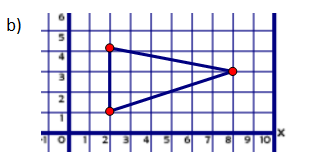Perimeter = ______ units 2.5 The student demonstrates no major errors or omissions regarding the simpler details and processes (Score 2.0 content) and partial knowledge of the more complex ideas and processes (Score 3.0 content). Progressing The student demonstrates no major errors or omissions regarding the simpler details and processes but exhibits major errors or omissions regarding the more complex ideas and processes (Score 3.0 content). The student can recognize and recall terminology to answer questions. The student can compute the perimeter or area of a figure on a coordinate plane without needing to use the distance formula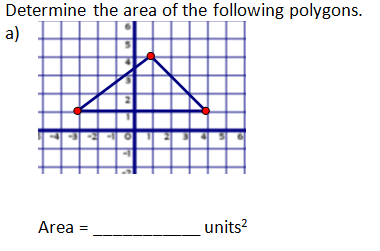1.5 The student demonstrates partial knowledge of the simpler details and processes (Score 2.0 content) but exhibits major errors or omissions regarding the more complex ideas and procedures (Score 3.0 content). Beginning With help, the student demonstrates a partial understanding of some of the simpler details and processes (Score 2.0 content) and some of the more complex ideas and processes (Score 3.0 content). - 0.5 With help, the student demonstrates a partial understanding of some of the simpler details and processes (Score 2.0 content) but not the more complex ideas and processes (Score 3.0 content). 0.0 Even with help, the student demonstrates no understanding or skill. -

## Resources

Vocabulary

Websites

#### MAT-HS.G-MG.01

 MAT-HS Targeted Standards(G) Concept: Geometry(MG) Domain: Modeling with GeometryCluster: Apply geometric concepts in modeling situations MAT-HS.G-MG.01 Use geometric shapes, their measures, and their properties to describe objects (e.g., modeling a tree trunk or a human torso as a cylinder).

## Student Learning Targets:

• I can
• I can

### Reasoning Targets

• I can determine an appropriate geometric model that can be used to solve a problem.
• I can use geometric shapes, their measures, and their properties to describe objects.

### Skills (Performance) Targets

• I can use geometric models to find solutions to problems in mathematics and other disciplines.

• I can
• I can

## Proficiency Scale

 Score Description Sample Activity 4.0 In addition to Score 3.0, the student demonstrates in-depth inferences and applications regarding more complex material that go beyond end of instruction expectations. - 3.5 In addition to Score 3.0 performance, the student demonstrates in-depth inferences and applications regarding the more complex content with partial success. 3.0 “The Standard.” The student demonstrates no major errors or omissions regarding any of the information and processes that were end of instruction expectations. - 2.5 The student demonstrates no major errors or omissions regarding the simpler details and processes (Score 2.0 content) and partial knowledge of the more complex ideas and processes (Score 3.0 content). 2.0 The student demonstrates no major errors or omissions regarding the simpler details and processes but exhibits major errors or omissions regarding the more complex ideas and processes (Score 3.0 content). - 1.5 The student demonstrates partial knowledge of the simpler details and processes (Score 2.0 content) but exhibits major errors or omissions regarding the more complex ideas and procedures (Score 3.0 content). 1.0 With help, the student demonstrates a partial understanding of some of the simpler details and processes (Score 2.0 content) and some of the more complex ideas and processes (Score 3.0 content). - 0.5 With help, the student demonstrates a partial understanding of some of the simpler details and processes (Score 2.0 content) but not the more complex ideas and processes (Score 3.0 content). 0.0 Even with help, the student demonstrates no understanding or skill. -

## Resources

 Web Vocab

#### MAT-HS.G-MG.02

 MAT-HS Targeted Standards(G) Concept: Geometry(MG) Domain: Modeling with GeometryCluster: Apply geometric concepts in modeling situations MAT-HS.G-MG.02 Apply concepts of density based on area and volume in modeling situations (e.g., persons per square mile, BTUs per cubic foot).

## Student Learning Targets:

• I can
• I can

### Reasoning Targets

• I can determine an appropriate geometric model that can be used to solve a problem.

### Skills (Performance) Targets

• I can use geometric models to find solutions to problems in mathematics and other disciplines.
• I can use the concept of density when referring to situations involving area and volume models, such as persons per square mile.

• I can
• I can

## Proficiency Scale

 Score Description Sample Activity 4.0 In addition to Score 3.0, the student demonstrates in-depth inferences and applications regarding more complex material that go beyond end of instruction expectations. - 3.5 In addition to Score 3.0 performance, the student demonstrates in-depth inferences and applications regarding the more complex content with partial success. 3.0 “The Standard.” The student demonstrates no major errors or omissions regarding any of the information and processes that were end of instruction expectations. - 2.5 The student demonstrates no major errors or omissions regarding the simpler details and processes (Score 2.0 content) and partial knowledge of the more complex ideas and processes (Score 3.0 content). 2.0 The student demonstrates no major errors or omissions regarding the simpler details and processes but exhibits major errors or omissions regarding the more complex ideas and processes (Score 3.0 content). - 1.5 The student demonstrates partial knowledge of the simpler details and processes (Score 2.0 content) but exhibits major errors or omissions regarding the more complex ideas and procedures (Score 3.0 content). 1.0 With help, the student demonstrates a partial understanding of some of the simpler details and processes (Score 2.0 content) and some of the more complex ideas and processes (Score 3.0 content). - 0.5 With help, the student demonstrates a partial understanding of some of the simpler details and processes (Score 2.0 content) but not the more complex ideas and processes (Score 3.0 content). 0.0 Even with help, the student demonstrates no understanding or skill. -

## Resources

 Web Vocab

#### MAT-HS.G-MG.03

 MAT-HS Targeted Standards(G) Concept: Geometry(MG) Domain: Modeling with GeometryCluster: Apply geometric concepts in modeling situations MAT-HS.G-MG.03 Apply geometric methods to solve design problems (e.g., designing an object or structure to satisfy physical constraints or minimize cost; working with typographic grid systems based on ratios).

## Student Learning Targets:

• I can
• I can

### Reasoning Targets

• I can determine an appropriate geometric model that can be used to solve a problem.

### Skills (Performance) Targets

• I can use geometric models to find solutions to problems in mathematics and other disciplines.
• I can solve problems by designing an object or structure that satisfies certain constraints, such as minimizing cost or the enlargement of a picture using a grid, ratios, and proportions.

• I can
• I can

## Proficiency Scale

 Score Description Sample Activity 4.0 In addition to Score 3.0, the student demonstrates in-depth inferences and applications regarding more complex material that go beyond end of instruction expectations. - 3.5 In addition to Score 3.0 performance, the student demonstrates in-depth inferences and applications regarding the more complex content with partial success. 3.0 “The Standard.” The student demonstrates no major errors or omissions regarding any of the information and processes that were end of instruction expectations. - 2.5 The student demonstrates no major errors or omissions regarding the simpler details and processes (Score 2.0 content) and partial knowledge of the more complex ideas and processes (Score 3.0 content). 2.0 The student demonstrates no major errors or omissions regarding the simpler details and processes but exhibits major errors or omissions regarding the more complex ideas and processes (Score 3.0 content). - 1.5 The student demonstrates partial knowledge of the simpler details and processes (Score 2.0 content) but exhibits major errors or omissions regarding the more complex ideas and procedures (Score 3.0 content). 1.0 With help, the student demonstrates a partial understanding of some of the simpler details and processes (Score 2.0 content) and some of the more complex ideas and processes (Score 3.0 content). - 0.5 With help, the student demonstrates a partial understanding of some of the simpler details and processes (Score 2.0 content) but not the more complex ideas and processes (Score 3.0 content). 0.0 Even with help, the student demonstrates no understanding or skill. -

## Resources

 Web Vocab

#### MAT-HS.G-SRT.01

 MAT-HS Targeted Standards(G) Concept: Geometry(SRT) Domain: Similarity, Right Triangle, and TrigonometryCluster: Understand similarity in terms of similarity transformations MAT-HS.G-SRT.01 Verify experimentally the properties of dilations given by a center and a scale factor. a. A dilation takes a line not passing through the center of the dilation to a parallel line, and leaves a line passing through the center unchanged. b. The dilation of a line segment is longer or shorter in the ratio given by the scale factor.

## Student Learning Targets:

• I can
• I can

• I can
• I can

### Skills (Performance) Targets

• I can verify experimentally the properties of dilations given by a center and a scale factor.
• I can verify experimentally that a dilation takes a line not passing through the center of the dilation to a parallel line, and leaves a line passing through the center unchanged.
• I can verify experimentally, that when performing dilations of a line segment, the image segment becomes longer or shorter based on the ratio given by the scale factor.

• I can
• I can

## Proficiency Scale

 Score Description Sample Activity 4.0 In addition to Score 3.0, the student demonstrates in-depth inferences and applications regarding more complex material that go beyond end of instruction expectations. - 3.5 In addition to Score 3.0 performance, the student demonstrates in-depth inferences and applications regarding the more complex content with partial success. 3.0 “The Standard.” The student demonstrates no major errors or omissions regarding any of the information and processes that were end of instruction expectations. - 2.5 The student demonstrates no major errors or omissions regarding the simpler details and processes (Score 2.0 content) and partial knowledge of the more complex ideas and processes (Score 3.0 content). 2.0 The student demonstrates no major errors or omissions regarding the simpler details and processes but exhibits major errors or omissions regarding the more complex ideas and processes (Score 3.0 content). - 1.5 The student demonstrates partial knowledge of the simpler details and processes (Score 2.0 content) but exhibits major errors or omissions regarding the more complex ideas and procedures (Score 3.0 content). 1.0 With help, the student demonstrates a partial understanding of some of the simpler details and processes (Score 2.0 content) and some of the more complex ideas and processes (Score 3.0 content). - 0.5 With help, the student demonstrates a partial understanding of some of the simpler details and processes (Score 2.0 content) but not the more complex ideas and processes (Score 3.0 content). 0.0 Even with help, the student demonstrates no understanding or skill. -

## Resources

 Web Vocab

#### MAT-HS.G-SRT.02

 MAT-HS Targeted Standards(G) Concept: Geometry(SRT) Domain: Similarity, Right Triangle, and TrigonometryCluster: Understand similarity in terms of similarity transformations MAT-HS.G-SRT.02 Given two figures, use the definition of similarity in terms of similarity transformations to decide if they are similar; explain using similarity transformations the meaning of similarity for triangles as the equality of all corresponding pairs of angles and the proportionality of all corresponding pairs of sides.

## Student Learning Targets:

### Knowledge Targets

• I can develop the definition of similarity using the idea of dilation transformations.

### Reasoning Targets

• I can determine congruence and similarity among geometric objects.
• I can use similarity or congruence to solve for a missing side/angle.
• I can determine whether two figures are similar.
• I can explain similarity based on the equality of corresponding angles and the proportionality of corresponding sides.

• I can
• I can

• I can
• I can

## Proficiency Scale

 Score Description Sample Activity 4.0 In addition to Score 3.0, the student demonstrates in-depth inferences and applications regarding more complex material that go beyond end of instruction expectations. - 3.5 In addition to Score 3.0 performance, the student demonstrates in-depth inferences and applications regarding the more complex content with partial success. 3.0 “The Standard.” The student demonstrates no major errors or omissions regarding any of the information and processes that were end of instruction expectations. - 2.5 The student demonstrates no major errors or omissions regarding the simpler details and processes (Score 2.0 content) and partial knowledge of the more complex ideas and processes (Score 3.0 content). 2.0 The student demonstrates no major errors or omissions regarding the simpler details and processes but exhibits major errors or omissions regarding the more complex ideas and processes (Score 3.0 content). - 1.5 The student demonstrates partial knowledge of the simpler details and processes (Score 2.0 content) but exhibits major errors or omissions regarding the more complex ideas and procedures (Score 3.0 content). 1.0 With help, the student demonstrates a partial understanding of some of the simpler details and processes (Score 2.0 content) and some of the more complex ideas and processes (Score 3.0 content). - 0.5 With help, the student demonstrates a partial understanding of some of the simpler details and processes (Score 2.0 content) but not the more complex ideas and processes (Score 3.0 content). 0.0 Even with help, the student demonstrates no understanding or skill. -

## Resources

 Web Vocab

#### MAT-HS.G-SRT.03

 MAT-HS Targeted Standards(G) Concept: Geometry(SRT) Domain: Similarity, Right Triangle, and TrigonometryCluster: Understand similarity in terms of similarity transformations MAT-HS.G-SRT.03 Use the properties of similarity transformations to establish the AA criterion for two triangles to be similar.

## Student Learning Targets:

• I can
• I can

• I can
• I can

### Skills (Performance) Targets

• I can use the properties of similarity transformations to prove triangles are similar by AA criterion.

• I can
• I can

## Proficiency Scale

 Score Description Sample Activity 4.0 In addition to Score 3.0, the student demonstrates in-depth inferences and applications regarding more complex material that go beyond end of instruction expectations. - 3.5 In addition to Score 3.0 performance, the student demonstrates in-depth inferences and applications regarding the more complex content with partial success. 3.0 “The Standard.” The student demonstrates no major errors or omissions regarding any of the information and processes that were end of instruction expectations. - 2.5 The student demonstrates no major errors or omissions regarding the simpler details and processes (Score 2.0 content) and partial knowledge of the more complex ideas and processes (Score 3.0 content). 2.0 The student demonstrates no major errors or omissions regarding the simpler details and processes but exhibits major errors or omissions regarding the more complex ideas and processes (Score 3.0 content). - 1.5 The student demonstrates partial knowledge of the simpler details and processes (Score 2.0 content) but exhibits major errors or omissions regarding the more complex ideas and procedures (Score 3.0 content). 1.0 With help, the student demonstrates a partial understanding of some of the simpler details and processes (Score 2.0 content) and some of the more complex ideas and processes (Score 3.0 content). - 0.5 With help, the student demonstrates a partial understanding of some of the simpler details and processes (Score 2.0 content) but not the more complex ideas and processes (Score 3.0 content). 0.0 Even with help, the student demonstrates no understanding or skill. -

## Resources

 Web Vocab

#### MAT-HS.G-SRT.04

 MAT-HS Targeted Standards(G) Concept: Geometry(SRT) Domain: Similarity, Right Triangle, and TrigonometryCluster: Prove theorems involving similarity MAT-HS.G-SRT.04 Prove theorems about triangles. Theorems include: a line parallel to one side of a triangle divides the other two proportionally, and conversely; the Pythagorean Theorem proved using triangle similarity.

## Student Learning Targets:

• I can
• I can

• I can
• I can

### Skills (Performance) Targets

• I can use AAA, SAS, SSS similarity theorems to prove triangles are similar.
• I can use triangle similarity to prove other theorems about triangles.
• I can prove a line parallel to one side of a triangle divides the other two proportionally, and it’s converse.
• I can prove the Pythagorean Theorem using triangle similarity.

• I can
• I can

## Proficiency Scale

 Score Description Sample Activity 4.0 In addition to Score 3.0, the student demonstrates in-depth inferences and applications regarding more complex material that go beyond end of instruction expectations. - 3.5 In addition to Score 3.0 performance, the student demonstrates in-depth inferences and applications regarding the more complex content with partial success. 3.0 “The Standard.” The student demonstrates no major errors or omissions regarding any of the information and processes that were end of instruction expectations. - 2.5 The student demonstrates no major errors or omissions regarding the simpler details and processes (Score 2.0 content) and partial knowledge of the more complex ideas and processes (Score 3.0 content). 2.0 The student demonstrates no major errors or omissions regarding the simpler details and processes but exhibits major errors or omissions regarding the more complex ideas and processes (Score 3.0 content). - 1.5 The student demonstrates partial knowledge of the simpler details and processes (Score 2.0 content) but exhibits major errors or omissions regarding the more complex ideas and procedures (Score 3.0 content). 1.0 With help, the student demonstrates a partial understanding of some of the simpler details and processes (Score 2.0 content) and some of the more complex ideas and processes (Score 3.0 content). - 0.5 With help, the student demonstrates a partial understanding of some of the simpler details and processes (Score 2.0 content) but not the more complex ideas and processes (Score 3.0 content). 0.0 Even with help, the student demonstrates no understanding or skill. -

## Resources

 Web Vocab

#### MAT-HS.G-SRT.05

 MAT-HS Targeted Standards(G) Concept: Geometry(SRT) Domain: Similarity, Right Triangle, and TrigonometryCluster: Prove theorems involving similarity MAT-HS.G-SRT.05 Use congruence and similarity criteria for triangles to solve problems and to prove relationships in geometric figures.

## Student Learning Targets:

• I can
• I can

### Reasoning Targets

• I can determine congruence and similarity among geometric objects.
• I can use similarity or congruence to solve for a missing side/angle.

### Skills (Performance) Targets

• I can use similarity and congruence criteria to solve real world problems.
• I can use congruence and similarity to prove relationships involving geometric figures.

• I can
• I can

## Proficiency Scale

 Score Description Sample Activity 4.0 In addition to Score 3.0, the student demonstrates in-depth inferences and applications regarding more complex material that go beyond end of instruction expectations. - 3.5 In addition to Score 3.0 performance, the student demonstrates in-depth inferences and applications regarding the more complex content with partial success. 3.0 “The Standard.” The student demonstrates no major errors or omissions regarding any of the information and processes that were end of instruction expectations. - 2.5 The student demonstrates no major errors or omissions regarding the simpler details and processes (Score 2.0 content) and partial knowledge of the more complex ideas and processes (Score 3.0 content). 2.0 The student demonstrates no major errors or omissions regarding the simpler details and processes but exhibits major errors or omissions regarding the more complex ideas and processes (Score 3.0 content). - 1.5 The student demonstrates partial knowledge of the simpler details and processes (Score 2.0 content) but exhibits major errors or omissions regarding the more complex ideas and procedures (Score 3.0 content). 1.0 With help, the student demonstrates a partial understanding of some of the simpler details and processes (Score 2.0 content) and some of the more complex ideas and processes (Score 3.0 content). - 0.5 With help, the student demonstrates a partial understanding of some of the simpler details and processes (Score 2.0 content) but not the more complex ideas and processes (Score 3.0 content). 0.0 Even with help, the student demonstrates no understanding or skill. -

## Resources

 Web Vocab

#### MAT-HS.G-SRT.05.a

 MAT-HS Targeted Standards(G) Concept: Geometry(SRT) Domain: Similarity, Right Triangle, and TrigonometryCluster: Prove theorems involving similarity MAT-HS.G-SRT.05.a Use congruence to solve problems in geometric figures.

• I can
• I can

• I can
• I can

• I can
• I can

• I can
• I can

## Proficiency Scale

 Score Description Sample Activity Advanced In addition to Score 3.0, the student demonstrates in-depth inferences and applications regarding more complex material that go beyond end of instruction expectations. Error analysis (explain why the reasoning of others is incorrect) Explain why the answer is incorrect and give the correct answer.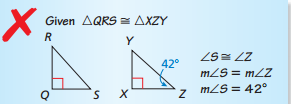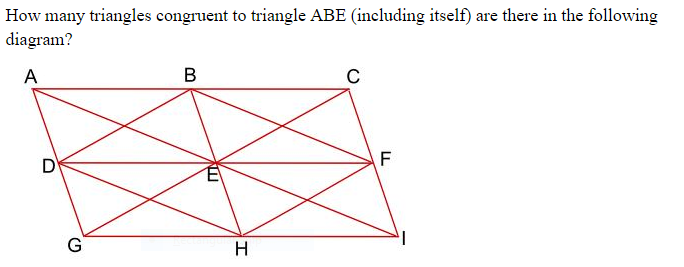3.5 In addition to Score 3.0 performance, the student demonstrates in-depth inferences and applications regarding the more complex content with partial success. Proficient “The Standard.” The student demonstrates no major errors or omissions regarding any of the information and processes that were end of instruction expectations.    Use congruence to solve problems.    Use congruence to show relationships in geometric figures (using CPCTC) Two door stops have cross sections that are right triangles.  They both have a 200 angle and the length of the side between the 900 and the 200 angles are equal.  Are the cross sections congruent?  Explain.   Solve for x and y: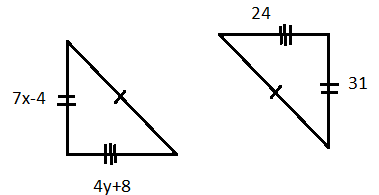2.5 The student demonstrates no major errors or omissions regarding the simpler details and processes (Score 2.0 content) and partial knowledge of the more complex ideas and processes (Score 3.0 content). Progressing The student demonstrates no major errors or omissions regarding the simpler details and processes but exhibits major errors or omissions regarding the more complex ideas and processes (Score 3.0 content). recognizes the congruence criteria for triangles (SAS, ASA, etc.) recognize and use specific terminology such as: Corresponding angles, corresponding sides, congruence statement Identity all the pairs of corresponding sides and angles. Then write a congruence statement.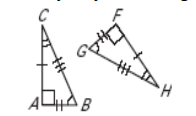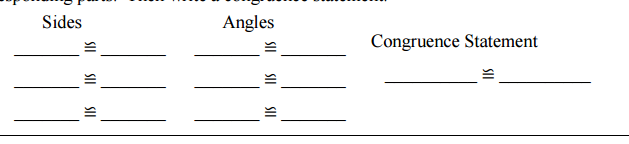1.5 The student demonstrates partial knowledge of the simpler details and processes (Score 2.0 content) but exhibits major errors or omissions regarding the more complex ideas and procedures (Score 3.0 content). Beginning With help, the student demonstrates a partial understanding of some of the simpler details and processes (Score 2.0 content) and some of the more complex ideas and processes (Score 3.0 content). - 0.5 With help, the student demonstrates a partial understanding of some of the simpler details and processes (Score 2.0 content) but not the more complex ideas and processes (Score 3.0 content). 0.0 Even with help, the student demonstrates no understanding or skill. -

## Resources

 Web Vocab

#### MAT-HS.G-SRT.05.b

 MAT-HS Targeted Standards(G) Concept: Geometry(SRT) Domain: Similarity, Right Triangle, and TrigonometryCluster: Prove theorems involving similarity MAT-HS.G-SRT.05.b Use similarity criteria to solve problems in geometric figures.

• I can
• I can

• I can
• I can

• I can
• I can

• I can
• I can

## Proficiency Scale

 Score Description Sample Activity Advanced In addition to Score 3.0, the student demonstrates in-depth inferences and applications regarding more complex material that go beyond end of instruction expectations.3.5 In addition to Score 3.0 performance, the student demonstrates in-depth inferences and applications regarding the more complex content with partial success. Proficient “The Standard.” The student demonstrates no major errors or omissions regarding any of the information and processes that were end of instruction expectations. use similarity properties and proportions to solve for missing measurements use similarity to prove relationships in geometric figures Find the height of the tree.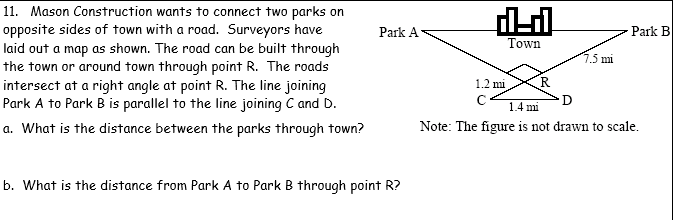2.5 The student demonstrates no major errors or omissions regarding the simpler details and processes (Score 2.0 content) and partial knowledge of the more complex ideas and processes (Score 3.0 content). Progressing The student demonstrates no major errors or omissions regarding the simpler details and processes but exhibits major errors or omissions regarding the more complex ideas and processes (Score 3.0 content). can solve provided proportionality problems can recognize vocabulary such as congruence, similarity, scale factor, ratio and proportion recognizes the similarity criteria for triangles (SAS, SSS, AA) Identify if the given triangles are similar. If they are similar, give the scale factor.Solve for x.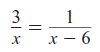1.5 The student demonstrates partial knowledge of the simpler details and processes (Score 2.0 content) but exhibits major errors or omissions regarding the more complex ideas and procedures (Score 3.0 content). Beginning With help, the student demonstrates a partial understanding of some of the simpler details and processes (Score 2.0 content) and some of the more complex ideas and processes (Score 3.0 content). - 0.5 With help, the student demonstrates a partial understanding of some of the simpler details and processes (Score 2.0 content) but not the more complex ideas and processes (Score 3.0 content). 0.0 Even with help, the student demonstrates no understanding or skill. -

## Resources

 Web Vocab

#### MAT-HS.G-SRT.05.c

 MAT-HS Targeted Standards(G) Concept: Geometry(SRT) Domain: Similarity, Right Triangle, and TrigonometryCluster: Prove theorems involving similarity MAT-HS.G-SRT.05.c Use similarity criteria to prove relationships in geometric figures.

• I can
• I can

• I can
• I can

• I can
• I can

• I can
• I can

## Proficiency Scale

 Score Description Sample Activity Advanced In addition to Score 3.0, the student demonstrates in-depth inferences and applications regarding more complex material that go beyond end of instruction expectations.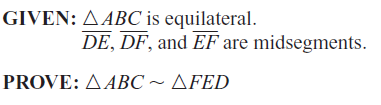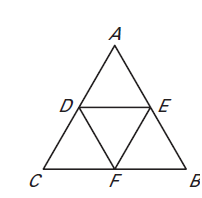3.5 In addition to Score 3.0 performance, the student demonstrates in-depth inferences and applications regarding the more complex content with partial success. Proficient “The Standard.” The student demonstrates no major errors or omissions regarding any of the information and processes that were end of instruction expectations. Use AA, SSS, and SAS Similarity Theorems to prove triangles similar Justify conclusions using valid mathematical arguments. Sketch the triangles using the given description. Explain/Justify whether the two triangles can be similar.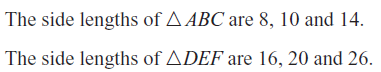2.5 The student demonstrates no major errors or omissions regarding the simpler details and processes (Score 2.0 content) and partial knowledge of the more complex ideas and processes (Score 3.0 content). Progressing The student demonstrates no major errors or omissions regarding the simpler details and processes but exhibits major errors or omissions regarding the more complex ideas and processes (Score 3.0 content). recognize vocabulary such as similarity, corresponding sides, and proportional recognizes the similarity criteria for triangles (SAS, AA, SSS) Are the triangles similar? If so, state the similarity and the postulate or theorem that justifies your answer.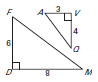1.5 The student demonstrates partial knowledge of the simpler details and processes (Score 2.0 content) but exhibits major errors or omissions regarding the more complex ideas and procedures (Score 3.0 content). Beginning With help, the student demonstrates a partial understanding of some of the simpler details and processes (Score 2.0 content) and some of the more complex ideas and processes (Score 3.0 content). - 0.5 With help, the student demonstrates a partial understanding of some of the simpler details and processes (Score 2.0 content) but not the more complex ideas and processes (Score 3.0 content). 0.0 Even with help, the student demonstrates no understanding or skill. -

## Resources

 Web Vocab

#### MAT-HS.G-SRT.06

 MAT-HS Targeted Standards(G) Concept: Geometry(SRT) Domain: Similarity, Right Triangle, and TrigonometryCluster: Define trigonometric ratios and solve problems involving right triangles. MAT-HS.G-SRT.06 Understand that by similarity, side ratios in right triangles are properties of the angles in the triangle, leading to definitions of trigonometric ratios for acute angles.

## Student Learning Targets:

### Knowledge Targets

• I can show that the side ratios are the same in similar right triangles, which leads to the definition of trigonometric ratios for acute angles.

• I can
• I can

• I can
• I can

• I can
• I can

## Proficiency Scale

 Score Description Sample Activity 4.0 In addition to Score 3.0, the student demonstrates in-depth inferences and applications regarding more complex material that go beyond end of instruction expectations. - 3.5 In addition to Score 3.0 performance, the student demonstrates in-depth inferences and applications regarding the more complex content with partial success. 3.0 “The Standard.” The student demonstrates no major errors or omissions regarding any of the information and processes that were end of instruction expectations. - 2.5 The student demonstrates no major errors or omissions regarding the simpler details and processes (Score 2.0 content) and partial knowledge of the more complex ideas and processes (Score 3.0 content). 2.0 The student demonstrates no major errors or omissions regarding the simpler details and processes but exhibits major errors or omissions regarding the more complex ideas and processes (Score 3.0 content). - 1.5 The student demonstrates partial knowledge of the simpler details and processes (Score 2.0 content) but exhibits major errors or omissions regarding the more complex ideas and procedures (Score 3.0 content). 1.0 With help, the student demonstrates a partial understanding of some of the simpler details and processes (Score 2.0 content) and some of the more complex ideas and processes (Score 3.0 content). - 0.5 With help, the student demonstrates a partial understanding of some of the simpler details and processes (Score 2.0 content) but not the more complex ideas and processes (Score 3.0 content). 0.0 Even with help, the student demonstrates no understanding or skill. -

## Resources

 Web Vocab

#### MAT-HS.G-SRT.07

 MAT-HS Targeted Standards(G) Concept: Geometry(SRT) Domain: Similarity, Right Triangle, and TrigonometryCluster: Define trigonometric ratios and solve problems involving right triangles. MAT-HS.G-SRT.07 Explain and use the relationship between the sine and cosine of complementary angles.

## Student Learning Targets:

### Knowledge Targets

• I can show that the side ratios are the same in similar right triangles, which leads to the definition of trigonometric ratios for acute angles.

### Reasoning Targets

• I can explain the relationship between the sine and cosine of complementary angles.

### Skills (Performance) Targets

• I can use the relationship between the sine and cosine of complementary angles.

• I can
• I can

## Proficiency Scale

 Score Description Sample Activity 4.0 In addition to Score 3.0, the student demonstrates in-depth inferences and applications regarding more complex material that go beyond end of instruction expectations. - 3.5 In addition to Score 3.0 performance, the student demonstrates in-depth inferences and applications regarding the more complex content with partial success. 3.0 “The Standard.” The student demonstrates no major errors or omissions regarding any of the information and processes that were end of instruction expectations. - 2.5 The student demonstrates no major errors or omissions regarding the simpler details and processes (Score 2.0 content) and partial knowledge of the more complex ideas and processes (Score 3.0 content). 2.0 The student demonstrates no major errors or omissions regarding the simpler details and processes but exhibits major errors or omissions regarding the more complex ideas and processes (Score 3.0 content). - 1.5 The student demonstrates partial knowledge of the simpler details and processes (Score 2.0 content) but exhibits major errors or omissions regarding the more complex ideas and procedures (Score 3.0 content). 1.0 With help, the student demonstrates a partial understanding of some of the simpler details and processes (Score 2.0 content) and some of the more complex ideas and processes (Score 3.0 content). - 0.5 With help, the student demonstrates a partial understanding of some of the simpler details and processes (Score 2.0 content) but not the more complex ideas and processes (Score 3.0 content). 0.0 Even with help, the student demonstrates no understanding or skill. -

## Resources

 Web Vocab

#### MAT-HS.G-SRT.08

 MAT-HS Targeted Standards(G) Concept: Geometry(SRT) Domain: Similarity, Right Triangle, and TrigonometryCluster: Define trigonometric ratios and solve problems involving right triangles MAT-HS.G-SRT.08 Use trigonometric ratios and the Pythagorean Theorem to solve right triangles in applied problems.

## Student Learning Targets:

### Knowledge Targets

• I can identify the hypotenuse and legs of a right triangle.
• I can choose the correct trigonometric ratio given a right triangle.
• I can identify the appropriate special right triangle.

### Reasoning Targets

• I can identify the hypotenuse and legs of a right triangle.
• I can choose the correct trigonometric ratio given a right triangle.
• I can identify the appropriate special right triangle.

### Skills (Performance) Targets

• I can apply trigonometric ratios and the Pythagorean Theorem to solve real world problems involving right triangles.

• I can
• I can

## Proficiency Scale

 Score Description Sample Activity 4.0 In addition to Score 3.0, the student demonstrates in-depth inferences and applications regarding more complex material that go beyond end of instruction expectations. - 3.5 In addition to Score 3.0 performance, the student demonstrates in-depth inferences and applications regarding the more complex content with partial success. 3.0 “The Standard.” The student demonstrates no major errors or omissions regarding any of the information and processes that were end of instruction expectations. - 2.5 The student demonstrates no major errors or omissions regarding the simpler details and processes (Score 2.0 content) and partial knowledge of the more complex ideas and processes (Score 3.0 content). 2.0 The student demonstrates no major errors or omissions regarding the simpler details and processes but exhibits major errors or omissions regarding the more complex ideas and processes (Score 3.0 content). - 1.5 The student demonstrates partial knowledge of the simpler details and processes (Score 2.0 content) but exhibits major errors or omissions regarding the more complex ideas and procedures (Score 3.0 content). 1.0 With help, the student demonstrates a partial understanding of some of the simpler details and processes (Score 2.0 content) and some of the more complex ideas and processes (Score 3.0 content). - 0.5 With help, the student demonstrates a partial understanding of some of the simpler details and processes (Score 2.0 content) but not the more complex ideas and processes (Score 3.0 content). 0.0 Even with help, the student demonstrates no understanding or skill. -

## Resources

 Web Vocab

#### MAT-HS.G-SRT.08.a

 MAT-HS Targeted Standards(G) Concept: Geometry(SRT) Domain: Similarity, Right Triangle, and TrigonometryCluster: Define trigonometric ratios and solve problems involving right triangles MAT-HS.G-SRT.08.a Use special right triangles (30°-60°-90° and 45°-45°-90°), trigonometric ratios and the Pythagorean Theorem to solve right triangles in applied problems.   (Mastery of the Pythagorean Theorem is an 8th grade standard.)

• I can
• I can

• I can
• I can

• I can
• I can

• I can
• I can

## Proficiency Scale

 Score Description Sample Activity Advanced In addition to Score 3.0, the student demonstrates in-depth inferences and applications regarding more complex material that go beyond end of instruction expectations. The student will be able to solve complex, multi step trigonometry and Pythagorean Theorem problems in applied situations.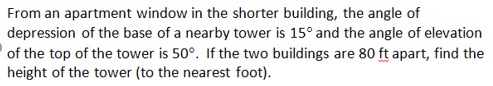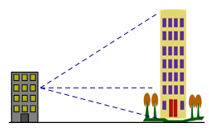3.5 In addition to Score 3.0 performance, the student demonstrates in-depth inferences and applications regarding the more complex content with partial success. Proficient “The Standard.” The student demonstrates no major errors or omissions regarding any of the information and processes that were end of instruction expectations. The student will be able to solve application problems involving trigonometry and Pythagorean Theorem.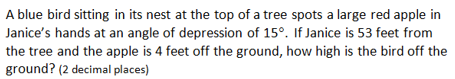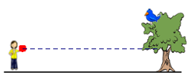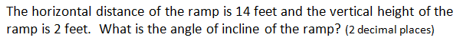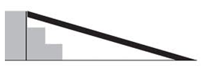The bottom of a ladder must be placed 3 feet from a wall.  The ladder is 12 feet long.  How far above the ground does the ladder touch the wall? 2.5 The student demonstrates no major errors or omissions regarding the simpler details and processes (Score 2.0 content) and partial knowledge of the more complex ideas and processes (Score 3.0 content). Progressing The student demonstrates no major errors or omissions regarding the simpler details and processes but exhibits major errors or omissions regarding the more complex ideas and processes (Score 3.0 content). The student can recognize and recall terminology to answer questions        ·  Angle of elevation        · Angle of depression        · Hypotenuse and legs of a right triangle The student can set up trigonometric ratios and solve a basic trigonometry problem. The student can correctly draw and label a diagram modeling the situation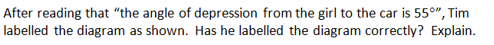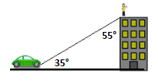Solve for x: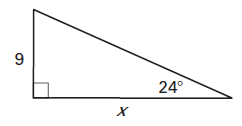1.5 The student demonstrates partial knowledge of the simpler details and processes (Score 2.0 content) but exhibits major errors or omissions regarding the more complex ideas and procedures (Score 3.0 content). Beginning With help, the student demonstrates a partial understanding of some of the simpler details and processes (Score 2.0 content) and some of the more complex ideas and processes (Score 3.0 content). - 0.5 With help, the student demonstrates a partial understanding of some of the simpler details and processes (Score 2.0 content) but not the more complex ideas and processes (Score 3.0 content). 0.0 Even with help, the student demonstrates no understanding or skill. -

## Resources

 Web Vocab

#### MAT-HS.G-SRT.08.b

 MAT-HS Targeted Standards(G) Concept: Geometry(SRT) Domain: Similarity, Right Triangle, and TrigonometryCluster: Define trigonometric ratios and solve problems involving right triangles MAT-HS.G-SRT.08.b  Use special right triangles (30°-60°-90° and 45°-45°-90°), trigonometric ratios and the Pythagorean Theorem to solve right triangles in applied problems.   (Mastery of the Pythagorean Theorem is an 8th grade standard.)

• I can
• I can

• I can
• I can

• I can
• I can

• I can
• I can

## Proficiency Scale

 Score Description Sample Activity Advanced In addition to Score 3.0, the student demonstrates in-depth inferences and applications regarding more complex material that go beyond end of instruction expectations. - 3.5 In addition to Score 3.0 performance, the student demonstrates in-depth inferences and applications regarding the more complex content with partial success. Proficient “The Standard.” The student demonstrates no major errors or omissions regarding any of the information and processes that were end of instruction expectations. The student will know and apply special right triangle relationships and use them to solve application problems. The length of a diagonal of a square is 18 cm.  Find the exact perimeter of the square. 2.5 The student demonstrates no major errors or omissions regarding the simpler details and processes (Score 2.0 content) and partial knowledge of the more complex ideas and processes (Score 3.0 content). Progressing The student demonstrates no major errors or omissions regarding the simpler details and processes but exhibits major errors or omissions regarding the more complex ideas and processes (Score 3.0 content). The student can recognize and recall terminology to answer questions Special right triangle (30-60-90, 45-45-90) Exact value Short leg Long leg Hypotenuse Isosceles Leg   The student can simplify radical expressions In each diagram, find the length of AC: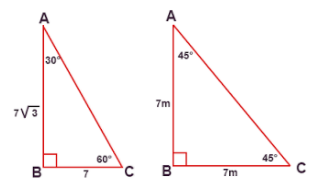1.5 The student demonstrates partial knowledge of the simpler details and processes (Score 2.0 content) but exhibits major errors or omissions regarding the more complex ideas and procedures (Score 3.0 content). Beginning With help, the student demonstrates a partial understanding of some of the simpler details and processes (Score 2.0 content) and some of the more complex ideas and processes (Score 3.0 content). - 0.5 With help, the student demonstrates a partial understanding of some of the simpler details and processes (Score 2.0 content) but not the more complex ideas and processes (Score 3.0 content). 0.0 Even with help, the student demonstrates no understanding or skill. -

## Resources

 Web Vocab

#### MAT-HS.G-SRT.09

 MAT-HS Targeted Standards(G) Concept: Geometry(SRT) Domain: Similarity, Right Triangle, and TrigonometryCluster: Define trigonometric ratios and solve problems involving right triangles. MAT-HS.G-SRT.09 Derive the formula A = ½ ab sin(C) for the area of a triangle by drawing an auxiliary line from a vertex perpendicular to the opposite side.

## Student Learning Targets:

• I can
• I can

• I can
• I can

### Skills (Performance) Targets

• I can for a triangle that is not a right triangle, draw an auxiliary line from a vertex, perpendicular to the opposite side and derive the formula, A=½ ab sin (C), for the area of a triangle, using the fact that the height of the triangle is, h=a sin(C).

• I can
• I can

## Proficiency Scale

 Score Description Sample Activity 4.0 In addition to Score 3.0, the student demonstrates in-depth inferences and applications regarding more complex material that go beyond end of instruction expectations. - 3.5 In addition to Score 3.0 performance, the student demonstrates in-depth inferences and applications regarding the more complex content with partial success. 3.0 “The Standard.” The student demonstrates no major errors or omissions regarding any of the information and processes that were end of instruction expectations. - 2.5 The student demonstrates no major errors or omissions regarding the simpler details and processes (Score 2.0 content) and partial knowledge of the more complex ideas and processes (Score 3.0 content). 2.0 The student demonstrates no major errors or omissions regarding the simpler details and processes but exhibits major errors or omissions regarding the more complex ideas and processes (Score 3.0 content). - 1.5 The student demonstrates partial knowledge of the simpler details and processes (Score 2.0 content) but exhibits major errors or omissions regarding the more complex ideas and procedures (Score 3.0 content). 1.0 With help, the student demonstrates a partial understanding of some of the simpler details and processes (Score 2.0 content) and some of the more complex ideas and processes (Score 3.0 content). - 0.5 With help, the student demonstrates a partial understanding of some of the simpler details and processes (Score 2.0 content) but not the more complex ideas and processes (Score 3.0 content). 0.0 Even with help, the student demonstrates no understanding or skill. -

## Resources

 Web Vocab

#### MAT-HS.G-SRT.10

 MAT-HS Targeted Standards(G) Concept: Geometry(SRT) Domain: Similarity, Right Triangle, and TrigonometryCluster: Apply trigonometry to general triangles MAT-HS.G-SRT.10 Prove the Laws of Sines and Cosines and use them to solve problems.

## Student Learning Targets:

• I can
• I can

• I can
• I can

### Skills (Performance) Targets

• I can prove the Laws of Sines and Cosines.
• I can use the Laws of Sines and Cosines to solve real world problems.

• I can
• I can

## Proficiency Scale

 Score Description Sample Activity 4.0 In addition to Score 3.0, the student demonstrates in-depth inferences and applications regarding more complex material that go beyond end of instruction expectations. - 3.5 In addition to Score 3.0 performance, the student demonstrates in-depth inferences and applications regarding the more complex content with partial success. 3.0 “The Standard.” The student demonstrates no major errors or omissions regarding any of the information and processes that were end of instruction expectations. - 2.5 The student demonstrates no major errors or omissions regarding the simpler details and processes (Score 2.0 content) and partial knowledge of the more complex ideas and processes (Score 3.0 content). 2.0 The student demonstrates no major errors or omissions regarding the simpler details and processes but exhibits major errors or omissions regarding the more complex ideas and processes (Score 3.0 content). - 1.5 The student demonstrates partial knowledge of the simpler details and processes (Score 2.0 content) but exhibits major errors or omissions regarding the more complex ideas and procedures (Score 3.0 content). 1.0 With help, the student demonstrates a partial understanding of some of the simpler details and processes (Score 2.0 content) and some of the more complex ideas and processes (Score 3.0 content). - 0.5 With help, the student demonstrates a partial understanding of some of the simpler details and processes (Score 2.0 content) but not the more complex ideas and processes (Score 3.0 content). 0.0 Even with help, the student demonstrates no understanding or skill. -

## Resources

 Web Vocab

#### MAT-HS.G-SRT.11

 MAT-HS Targeted Standards(G) Concept: Geometry(SRT) Domain: Similarity, Right Triangle, and TrigonometryCluster: Apply trigonometry to general triangles. MAT-HS.G-SRT.11 Understand and apply the Law of Sines and the Law of Cosines to find unknown measurements in right and non-right triangles (e.g., surveying problems, resultant forces).

## Student Learning Targets:

• I can
• I can

• I can
• I can

### Skills (Performance) Targets

• I can apply any method to find unknown measurements in right triangles.
• I can apply the Laws of Sines and Cosines to find unknown measurements in non-right triangles.

• I can
• I can

## Proficiency Scale

 Score Description Sample Activity 4.0 In addition to Score 3.0, the student demonstrates in-depth inferences and applications regarding more complex material that go beyond end of instruction expectations. - 3.5 In addition to Score 3.0 performance, the student demonstrates in-depth inferences and applications regarding the more complex content with partial success. 3.0 “The Standard.” The student demonstrates no major errors or omissions regarding any of the information and processes that were end of instruction expectations. - 2.5 The student demonstrates no major errors or omissions regarding the simpler details and processes (Score 2.0 content) and partial knowledge of the more complex ideas and processes (Score 3.0 content). 2.0 The student demonstrates no major errors or omissions regarding the simpler details and processes but exhibits major errors or omissions regarding the more complex ideas and processes (Score 3.0 content). - 1.5 The student demonstrates partial knowledge of the simpler details and processes (Score 2.0 content) but exhibits major errors or omissions regarding the more complex ideas and procedures (Score 3.0 content). 1.0 With help, the student demonstrates a partial understanding of some of the simpler details and processes (Score 2.0 content) and some of the more complex ideas and processes (Score 3.0 content). - 0.5 With help, the student demonstrates a partial understanding of some of the simpler details and processes (Score 2.0 content) but not the more complex ideas and processes (Score 3.0 content). 0.0 Even with help, the student demonstrates no understanding or skill. -

## Resources

 Web Vocab

Page:  1  2  3  4  5  6  (Next)
ALL